# 4.3. Southern Ocean Reentrant Channel Example¶

This example experiment simulates flow through a reentrant channel, crudely mimicking the Antartic Circumpolar Current. The fluid is forced by a zonal wind stress, $$\tau_x$$, that varies sinusoidally in the north-south direction and is constant in time, and by temperature relaxation at the surface and northern boundary. The grid is Cartesian and the Coriolis parameter $$f$$ is defined according to a mid-latitude beta-plane equation $$f(y) = f_{0}+\beta y$$ ; here we choose $$f_0 < 0$$ to place our domain in the Southern Hemisphere. A linear EOS is used with density only depending on T, and there is no sea ice.

Although important aspects of the of the Southern Ocean and Antarctic Circumpolar Current were realized in the early 20th Century (e.g., Sverdrup 1933 [Sve33]), understanding this system has been a major research focus in recent decades. Many significant breakthroughs in understanding its dynamics, role in the global ocean circulation, and role in the climate system have been achieved (e.g., Marshall and Radko 2003 [MR03]; Olbers and Visbeck 2004 [OV04]; Marshall and Speer 2012 [MS12]; Nikurashin and Vallis 2012 [NV12]; Armour et al. 2016 [AMS+16];Sallée 2018 [Sal18]). Much of this understanding came about using simple, idealized reentrant channel models in the spirit of the model described in this tutorial. The configuration here is fairly close to that employed in Abernathy et al. (2011) [AMF11] (using the MITgcm) with some important differences, such as our introduction of a deep north-south ridge.

We assume the reader is familiar with a basic MITgcm setup, as introduced in tutorial Barotropic Ocean Gyre and tutorial Baroclinic Ocean Gyre. Although the setup here is again quite idealized, we introduce many new features and capabilities of MITgcm. Novel aspects include using MITgcm packages to augment the physical modeling capabilities, discussion of partial cells to represent topography, and an introduction to the layers diagnostics package (/pkg/layers). Our initial focus is on running and comparing coarse-resolution solutions with and without activating the Gent-McWilliams (“GM”) (1990) [GM90] mesoscale eddy parameterization (/pkg/gmredi). As first noted in Danabashoglu et al. (1994) [DMG94], use of GM in coarse resolution models improves global temperature distribution, poleward and surface heat fluxes, and locations of deep-water formation (see also the Gent 2011 [Gen11] perspective on two decades GM usage in ocean models). At the end of this tutorial, we will describe how to increase resolution to an eddy-permitting regime, detailing the few necessary changes in code and parameters, and examine this high-resolution solution. In our discussion, our focus will be on highlighting how the representation of mesoscale eddies plays a significant role in governing the equilibrium state.

Below we describe the idealized configuration in detail (see Figure 4.10). The sinusoidal wind-stress variations are defined thus:

$\tau_x(y) = \tau_{0}\sin \left( \frac{y}{2 L_y} \pi \right),$

where $$L_{y}$$ is the lateral domain extent and $$\tau_0$$ is set to $$0.2 \text{ N m}^{-2}$$. Surface temperature restoring varies linearly from 10 oC at the northern boundary to -2 oC at the southern end. A wall is placed at the southern boundary of our domain, thus our setup is only reentrant in the east-west direction. Because MITgcm assumes a periodic domain in both the east-west and north-south directions, our southern wall effectively functions as a wall at the northern boundary as well. The full water column in the northern boundary is a “sponge layer”; relaxing temperature though the full water column will partially constrain the stratification, and in the eddy-permitting solution will absorb any eddies reaching the northern boundary (truly acting as a “sponge”). As shown in Figure 4.10, a north-south ridge runs through the bottom topography, which is otherwise flat with a depth $$H$$ of 3980 m. A sloping notch cuts through the middle of the ridge; in the latitude band where the notch exists, potential vorticity $$f/H$$ contours are unblocked, which permits a vigorous zonal barotropic jet. Shaved cells are used to represent the topography.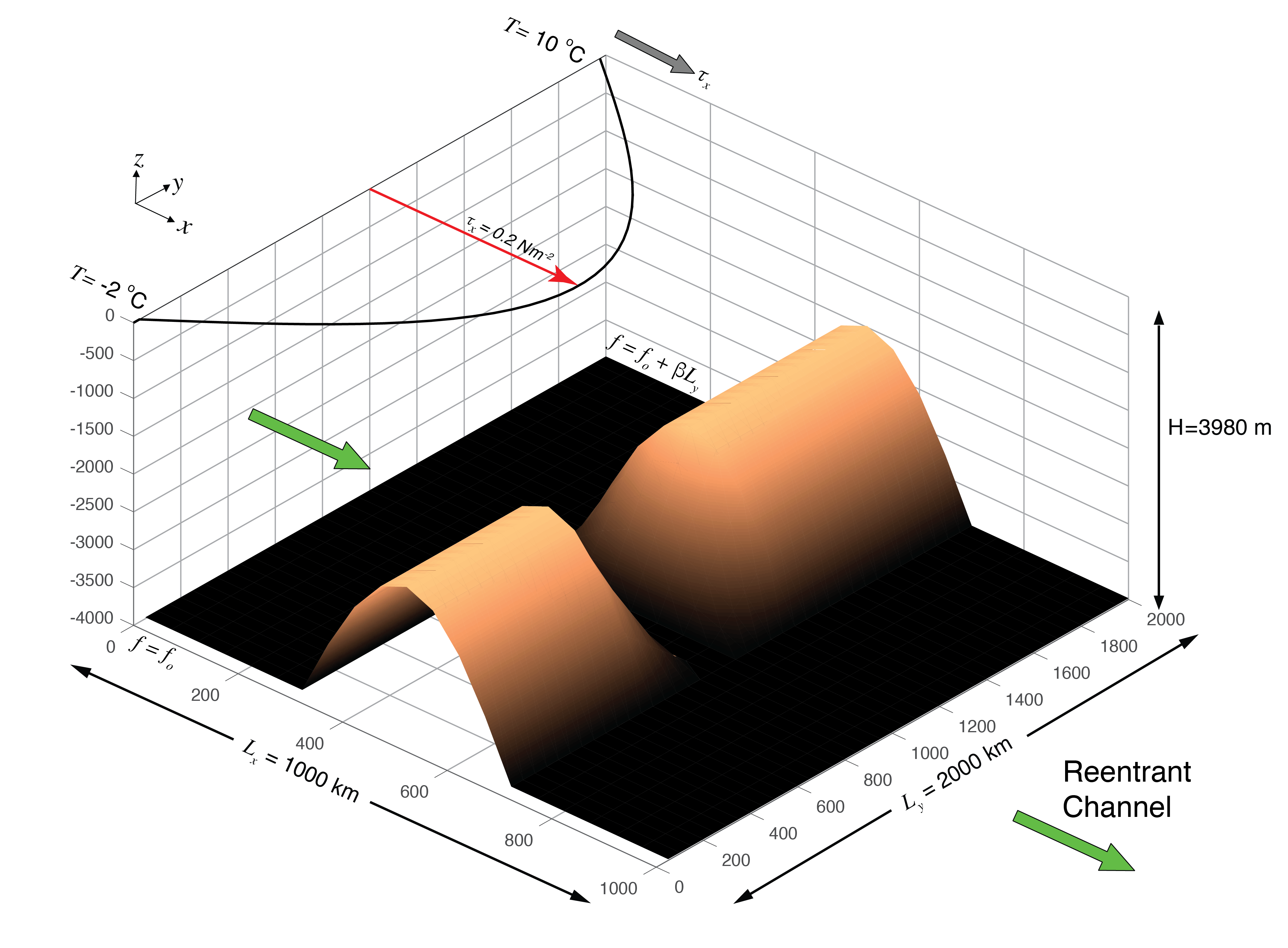Figure 4.10 Schematic of simulation domain, bottom topography, and wind-stress forcing function for the idealized reentrant channel numerical setup. A full-depth solid wall at $$y=$$ 0 is not shown; because MITgcm is also periodic in the north-south direction, this acts as a wall on the north boundary.¶

Similar to both tutorial Barotropic Ocean Gyre and tutorial Baroclinic Ocean Gyre, we use a linear equation of state which is a function of temperature only (temperature is our only model tracer field). Figure 4.11 shows initial conditions in temperature at the northern and southern end of the domain. Initial temperature decreases exponentially from the relaxation SST profile to -2 oC at depth $$H$$. Note that this same northern boundary profile is used to restore temperature in the model’s sponge layer, as discussed above.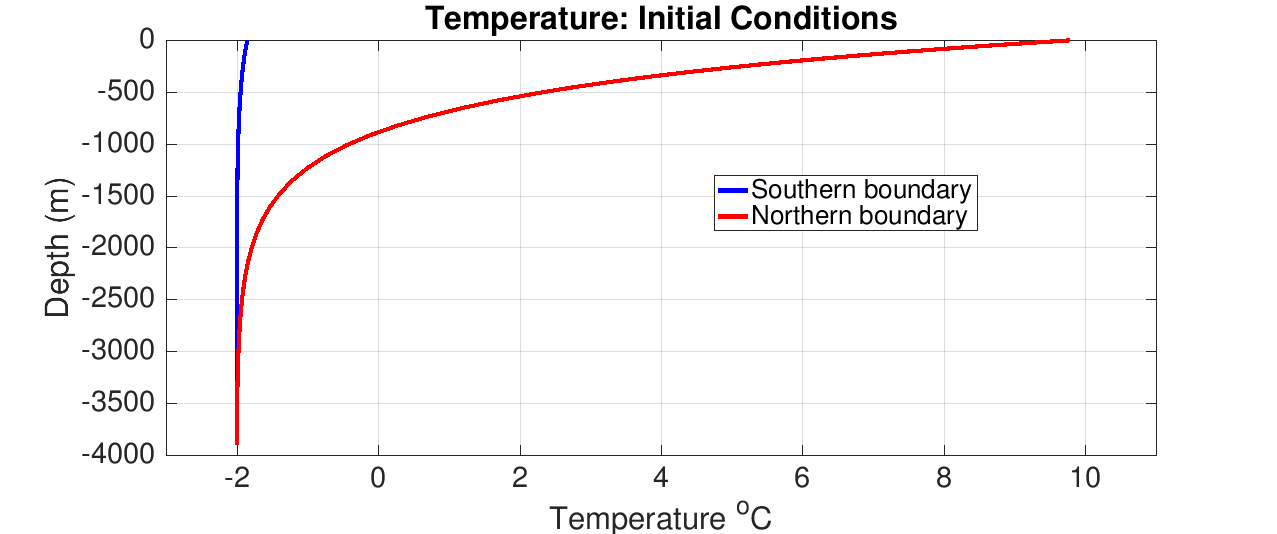Figure 4.11 Initial conditions in temperature at the northern and southern boundaries. Note this same northern boundary profile is used as relaxation temperature in the model’s sponge layer.¶

## 4.3.1. Equations Solved¶

The active set of equations solved is identical to those employed in tutorial Baroclinic Ocean Gyre (i.e., hydrostatic with an implicit linearized free surface), except here we use standard Cartesian geometry rather than spherical polar coordinates:

(4.21)$\begin{split}\frac{Du}{Dt} - fv + \frac{1}{\rho_c}\frac{\partial p'}{\partial x} + \nabla _h \cdot ( -A_{h} \nabla _h u ) + \frac{\partial}{\partial z} \left( -A_{z}\frac{\partial u}{\partial z} \right) &= \mathcal{F}_u \\ \frac{Dv}{Dt} + fu + \frac{1}{\rho_c}\frac{\partial p'}{\partial y} + \nabla _h \cdot ( -A_{h} \nabla _h v ) + \frac{\partial}{\partial z} \left( -A_{z}\frac{\partial v}{\partial z} \right) &= \mathcal{F}_v\end{split}$
$\frac{\partial \eta}{\partial t} + \nabla _h \cdot \left( H \vec{\widehat{\bf u}} \right) = 0$
(4.22)$\frac{D\theta}{Dt} + \nabla _h \cdot (-\kappa_{h} \nabla _h \theta) + \frac{\partial}{\partial z} \left( -\kappa_{z}\frac{\partial \theta}{\partial z} \right) = \mathcal{F}_\theta$
(4.23)$p^{\prime} = g\rho_{c} \eta + \int^{0}_{z} g \rho^{\prime} dz$

Forcing term $$\mathcal{F}_u$$ is applied as a source term in the model surface layer and zero in the interior, and source term $$\mathcal{F}_v$$ is zero everywhere. The forcing term $$\mathcal{F}_\theta$$ is applied as temperature relaxation in the surface layer and throughout the full depth in the two northern-most rows (in the coarse resolution setup) of the model domain.

## 4.3.2. Discrete Numerical Configuration¶

The coarse-resolution domain is discretized with a uniform Cartesian grid spacing in the horizontal set to $$\Delta x=\Delta y=50$$ km, so that there are 20 grid cells in the $$x$$ direction and 40 in the $$y$$ direction. There are 49 levels in the vertical, ranging from 5.5 m depth at the surface to 149 m at depth. An “optimal grid” vertical spacing here was generated using the hyperbolic tangent method of Stewart et al. (2017) [SHG+17], implemented in Python at https://github.com/kialstewart/vertical_grid_for_ocean_models, based on input parameters of ocean depth (4000 m), minimum (surface) depth (5 m), and maximum depth (150 m). In ocean modeling, it is generally advantageous to have finer resolution in the upper ocean (as was also done previously in tutorial Baroclinic Ocean Gyre), but note that the transition to deeper layers should be done gradually, in the interests of solution fidelity and stability. Although our topography is idealized, the topography is not a priori discretized to levels matching the vertical grid, and we make use of MITgcm’s ability to represent “partial cells” (see Section 2.11.6).

Otherwise, the numerical configuration is similar to that of tutorial Baroclinic Ocean Gyre), with an important difference: we use a high-order advection scheme (“7th order one-step method w/limiter”, tempAdvScheme parameter code 7) for potential temperature instead of center second-ordered differences (which is used in tutorials Barotropic Ocean Gyre and Baroclinic Ocean Gyre and is the model default). This will enable us to use the same numerical scheme in both coarse-resolution and eddy-permitting simulations. Note that this advection scheme does NOT use Adams-Bashforth time stepping for potential temperature, instead using its own time stepping scheme. The fixed flux form of the momentum equations are solved, as described in Section 2.14, with an implicit linear free surface (Section 2.4). Laplacian diffusion of tracers and momentum is employed. The pressure forces that drive the fluid motions, $$\partial_x p^\prime$$ and $$\partial_y p^\prime$$, are found by summing pressure due to surface elevation $$\eta$$ and the hydrostatic pressure, as discussed in Section 4.2.1. The sea-surface height is found by solving implicitly the 2-D (elliptic) surface pressure equation (see Section 2.4).

Additional changes in the numerical configuration for the eddy-permitting simulation are discussed in Section 4.3.5.2.

### 4.3.2.1. Numerical Stability Criteria¶

The numerical considerations behind our setup are not trivial. We do not wish the thermocline to be diffused away by numerics. Accordingly, we employ a vertical diffusivity acting on temperature typical of background values observed in the ocean, $$1 \times 10^{-5}$$ m2 s–1). We now examine numerical stability criteria to help choose and assess parameters for our coarse resolution study: parameters used in the eddy-permitting setup are discussed in Section 4.3.5.2.

We anticipate development of a large barotropic flow through the notch in the topographic ridge which will have implications for the length of the timestep we will be able to use. Let us consider the advective CFL condition (4.24) and the stability of inertial oscillations (4.25):

(4.24)$S_{\rm adv} = 2 \left( \frac{ |c_{\rm max}| \Delta t}{ \Delta x} \right) < 0.5 \text{ for stability}$
(4.25)$S_{\rm inert} = f {\Delta t} < 0.5 \text{ for stability}$

where $$|c_{\rm max}|$$ is the maximum horizontal velocity. We anticipate $$|c_{\rm max}|$$ of order ~ 1 ms-1. Note that barotropic currents of this speed over a jet of order ~ 100 km in lateral scale will result in a barotropic flow of the order of hundreds of Sverdups. At a resolution of 50 km, (4.24) then implies that the timestep must be less than 12000 s and (4.25) implies a timestep less than 3500 s. Here we make a conservative choice of $$\Delta t = 1000$$ s to keep $$f {\Delta t}$$ under 0.20.

How shall we set the horizontal viscosity? From the numerical stability criteria:

(4.26)$S_{\ Lh} = 4 A_{h} \Delta t \left( \frac{1}{{\Delta x}^2} + \frac{1}{{\Delta y}^2} \right) < 1.0 \text{ for stability}$

Note that the threshold in (4.26) is < 1.0 instead of < 0.6 due to our specification (in input/data) that momentum dissipation NOT be solved using Adams-Bashforth, as discussed below. With $$\Delta t = 1000$$ s, we can choose $$A_{h}$$ to be as large as order $$1 \times 10^{5}$$ m2 s–1. However, such a value would result in a very viscous solution. We anticipate a boundary current along the deep ridge and sloping notch on a scale given by Munk scaling:

(4.27)$M = \frac{2\pi}{\sqrt{3}} \left( \frac { A_{h} }{ \beta } \right)^{\frac{1}{3}}.$

We can set $$A_{h}$$ to as low as 100 m2 s–1 and still comfortably resolve the Munk boundary layer on our grid. However, guided by an ensemble of runs exploring parameter space, we found the solution with $$A_{h} = 100$$ s–1, while stable, was rather noisy. As a compromise, a value of $$A_{h} = 2000$$ m2 s–1 reduced solution noise whilst also controlling the strength of the barotropic current. This is the value used here. Also note with this choice $$A_{h} / \Delta x$$ gives a velocity scaling of 4 cm/s, a reasonable value.

Regarding the vertical viscosity, we choose to solve this term implicitly (Euler backward time-stepping) by setting implicitViscosity to .TRUE. in input/data, which results in no additional stability constraint on the model timestep (see Section 2.6). Otherwise, given that our vertical resolution is quite fine near the surface (approximately 5 m), the following stability criteria would have applied:

(4.28)$S_{\rm Lv} = 4 \frac{A_{v} \Delta t}{{\Delta z}^2} < 1.0 \text{ for stability}$

which effectively would limit our choice for $$A_{v}$$ to very small values. For simplicity, and given that away from the equator coarse resolution models are typically not very sensitive to the value of vertical viscosity, we pick a constant value of $$A_{v} = 3\times10^{-3}$$ m2 s–1 over the full domain, somewhere in between (in geometric mean sense) typical values found in the mixed layer ($$\sim 10^{-2}$$) and in the deep ocean ($$\sim 10^{-4}$$) (Roach et al. 2015 [RPBR15]) Note this implicit scheme is also used for vertical diffusion of tracers, for which it can also be used to represent convective adjustment (again, because it is unconditionally stable regardless of diffusivity value).

## 4.3.3. Configuration¶

The model configuration for this experiment resides under the directory verification/tutorial_reentrant_channel/.

The experiment files

contain the code customizations and parameter settings for this experiment. Below we describe these customizations in detail.

### 4.3.3.1. Compile-time Configuration¶

#### 4.3.3.1.1. File code/packages.conf¶

Listing 4.13 verification/tutorial_reentrant_channel/code/packages.conf
1#-- list of packages (or group of packages) to compile for this experiment:
2gfd
3gmredi
4rbcs
5layers
6diagnostics


In addition to the pre-defined standard package group gfd, we define four additional packages.

• Package pkg/gmredi (see GMREDI: Gent-McWilliams/Redi Eddy Parameterization): This implements the Gent and McWilliams parameterization (as first described in Gent and McWilliams 1990 [GM90]) of geostrophic eddies. This mixes along sloping neutral surfaces (here, just $$T$$ surfaces). It is used instead of large prescribed diffusivities aligned in the horizontal plane (parameter diffKh). In Section 4.3.5.1 we will illustrate the marked improvement in the solution resulting from the use of this parameterization.

• Package pkg/rbcs (see RBCS Package): The default MITgcm code library permits relaxation boundary conditions only at the ocean surface; in the setup here, we relax temperature over the full-depth $$xz$$ plane along our domain’s northern border. By including the pkg/rbcs code library in our model build, we can relax selected fields (tracers or horizontal velocities) in any 3-D location.

We also include two packages which augment MITgcm’s diagnostic capabilities.

• Package pkg/layers: This calculates the thickness and transport of layers of specified density (or temperature, or salinity; here, temperature and density are aligned because of our simple equation of state). Further explanation of pkg/layers parameter options and output is given below.

• Package pkg/diagnostics: This selects which fields to output, and at what frequencies. This was introduced in tutorial Baroclinic Ocean Gyre.

#### 4.3.3.1.2. File code/SIZE.h¶

Listing 4.14 verification/tutorial_reentrant_channel/code/SIZE.h
 1CBOP
2C    !ROUTINE: SIZE.h
3C    !INTERFACE:
4C    include SIZE.h
5C    !DESCRIPTION: \bv
6C     *==========================================================*
7C     | SIZE.h Declare size of underlying computational grid.
8C     *==========================================================*
9C     | The design here supports a three-dimensional model grid
10C     | with indices I,J and K. The three-dimensional domain
11C     | is comprised of nPx*nSx blocks (or tiles) of size sNx
12C     | along the first (left-most index) axis, nPy*nSy blocks
13C     | of size sNy along the second axis and one block of size
14C     | Nr along the vertical (third) axis.
15C     | Blocks/tiles have overlap regions of size OLx and OLy
16C     | along the dimensions that are subdivided.
17C     *==========================================================*
18C     \ev
19C
20C     Voodoo numbers controlling data layout:
21C     sNx :: Number of X points in tile.
22C     sNy :: Number of Y points in tile.
23C     OLx :: Tile overlap extent in X.
24C     OLy :: Tile overlap extent in Y.
25C     nSx :: Number of tiles per process in X.
26C     nSy :: Number of tiles per process in Y.
27C     nPx :: Number of processes to use in X.
28C     nPy :: Number of processes to use in Y.
29C     Nx  :: Number of points in X for the full domain.
30C     Ny  :: Number of points in Y for the full domain.
31C     Nr  :: Number of points in vertical direction.
32CEOP
33      INTEGER sNx
34      INTEGER sNy
35      INTEGER OLx
36      INTEGER OLy
37      INTEGER nSx
38      INTEGER nSy
39      INTEGER nPx
40      INTEGER nPy
41      INTEGER Nx
42      INTEGER Ny
43      INTEGER Nr
44      PARAMETER (
45     &           sNx =  20,
46     &           sNy =  10,
47     &           OLx =   4,
48     &           OLy =   4,
49     &           nSx =   1,
50     &           nSy =   4,
51     &           nPx =   1,
52     &           nPy =   1,
53     &           Nx  = sNx*nSx*nPx,
54     &           Ny  = sNy*nSy*nPy,
55     &           Nr  =   49)
56
57C     MAX_OLX :: Set to the maximum overlap region size of any array
58C     MAX_OLY    that will be exchanged. Controls the sizing of exch
59C                routine buffers.
60      INTEGER MAX_OLX
61      INTEGER MAX_OLY
62      PARAMETER ( MAX_OLX = OLx,
63     &            MAX_OLY = OLy )


Our model tile size is defined above to be 20 $$\times$$ 10 gridpoints, so four tiles (i.e., nSy =4) are required to span the full domain in $$y$$. Note that our overlap sizes (OLx, OLy) are set to 4 in this tutorial, as required by our choice of advection scheme (see discussion in Section 4.3.2.1 and Table 2.2 from which this required overlap can be obtained); in tutorial Baroclinic Ocean Gyre this was set to 2, which is the mimimum required for the default center second-ordered differences scheme. For this setup we will specify a reasonably high resolution in the vertical, using 49 levels.

#### 4.3.3.1.3. File code/LAYERS_SIZE.h¶

Listing 4.15 verification/tutorial_reentrant_channel/code/LAYERS_SIZE.h
 1C ======================================================================
2C * Compiled-in size options for the LAYERS package *
3C
4C  - Just as you have to define Nr in SIZE.h, you must define the number
5C    of vertical layers for isopycnal averaging so that the proper array
6C    sizes can be declared in the LAYERS.h header file.
7C
8C  - Variables -
9C      NLayers        :: the number of isopycnal layers (must match data.layers)
10C      FineGridFact   :: how many fine-grid cells per dF cell
11C      FineGridMax    :: the number of points in the finer vertical grid
12C                        used for interpolation
13C      layers_maxNum  :: max number of tracer fields used for layer averaging
14      INTEGER    Nlayers, FineGridFact, FineGridMax, layers_maxNum
15      PARAMETER( Nlayers = 37 )
16      PARAMETER( FineGridFact = 10 )
17      PARAMETER( FineGridMax = Nr * FineGridFact )
18      PARAMETER( layers_maxNum = 1 )


As noted above in this file’s comments, we must set the discrete number of layers to use in our diagnostic calculations. The model default is 20 layers. Here we set PARAMETER( Nlayers = 37 ) and so choose 37 layers. In making this choice, one needs to ensure sufficiently fine layer bounds in the density (or temperature) range of interest, while also possible to specify fairly coarse bounds in other density ranges. The specific temperatures defining layer bounds will be prescribed in input/data.layers

#### 4.3.3.1.4. File code/DIAGNOSTICS_SIZE.h¶

Listing 4.16 verification/tutorial_reentrant_channel/code/DIAGNOSTICS_SIZE.h
 1C     Diagnostics Array Dimension
2C     ---------------------------
3C     ndiagMax   :: maximum total number of available diagnostics
4C     numlists   :: maximum number of diagnostics list (in data.diagnostics)
5C     numperlist :: maximum number of active diagnostics per list (data.diagnostics)
6C     numLevels  :: maximum number of levels to write    (data.diagnostics)
7C     numDiags   :: maximum size of the storage array for active 2D/3D diagnostics
8C     nRegions   :: maximum number of regions (statistics-diagnostics)
9C     sizRegMsk  :: maximum size of the regional-mask (statistics-diagnostics)
10C     nStats     :: maximum number of statistics (e.g.: aver,min,max ...)
11C     diagSt_size:: maximum size of the storage array for statistics-diagnostics
12C Note : may need to increase "numDiags" when using several 2D/3D diagnostics,
13C  and "diagSt_size" (statistics-diags) since values here are deliberately small.
14      INTEGER    ndiagMax
15      INTEGER    numlists, numperlist, numLevels
16      INTEGER    numDiags
17      INTEGER    nRegions, sizRegMsk, nStats
18      INTEGER    diagSt_size
19      PARAMETER( ndiagMax = 500 )
20      PARAMETER( numlists = 10, numperlist = 50, numLevels=2*Nr )
21      PARAMETER( numDiags = 35*Nr )
22      PARAMETER( nRegions = 0 , sizRegMsk = 1 , nStats = 4 )
23      PARAMETER( diagSt_size = 10*Nr )


Here the parameter numDiags has been changed to allow a combination of up to 35 3-D diagnostic fields or 1715 (=35*49) 2-D fields.

### 4.3.3.2. Run-time Configuration¶

#### 4.3.3.2.1. File input/data¶

Listing 4.17 verification/tutorial_reentrant_channel/input/data
  1# Model parameters
2# Continuous equation parameters
3 &PARM01
4# Viscosity
5 viscAh=2000.,
6 viscAr=3.E-3,
7 implicitViscosity=.TRUE.,
8# Diffusivity and convection
9 diffKhT=0.,
10 diffKrT=1.E-5,
11 ivdc_kappa=1.,
12 implicitDiffusion=.TRUE.,
13# Coriolis parameter
14 f0=-1.363e-4,
15 beta=1.313e-11,
16 selectCoriScheme=1,
17# Density and equation of state
18# Temp only active tracer, no salinity
19 rhoConst=1035.,
20 rhoNil=1035.,
21 eosType='LINEAR',
22 tAlpha=2.E-4,
23 sBeta =0.E-4,
24 tRef= 49*5.,
25 saltStepping=.FALSE.,
26# activate partial cells
27 hFacMinDr=5.,
28 hFacMin=0.1,
29# free surface parameters
30 rigidLid=.FALSE.,
31 implicitFreeSurface=.TRUE.,
32 exactConserv=.TRUE.,
35 staggerTimeStep=.TRUE.,
36#---------------------------------------
37#- for non-GM coarse run, set horizontal diffusivity non-zero:
38# diffKhT=1000.,
39#
40#- for eddy-permitting run, uncomment the following:
41# viscC2Leith = 1.,
42# useFullLeith=.TRUE.,
43# viscAhGridMax = 0.5,
44# useSingleCpuIO=.TRUE.,
45#- and comment out above statement viscAh=2000.,
46 &
47
48# Elliptic solver parameters
49 &PARM02
50 cg2dTargetResidual=1.E-7,
51 cg2dMaxIters=1000,
52 &
53
54# Time stepping parameters
55 &PARM03
56 nIter0=0,
57 nTimeSteps=10,
58 deltaT=1000.0,
59 pChkptFreq=31104000.0,
60 chkptFreq=15552000.0,
61 dumpFreq=0,
62 monitorFreq=1200.,
63 monitorSelect=2,
64 tauThetaClimRelax=864000.,
65 momDissip_In_AB=.FALSE.,
66#---------------------------------------
67#- change monitor frequency for longer run:
68# monitorFreq=864000.,
69#
70#- nTimesteps for 30 yrs, coarse:
71# nTimeSteps=933120,
72#
73#- nTimesteps for 30 yrs, eddy-permitting:
74# nTimeSteps=3732480,
75#
76#- for eddy-permitting run, also need to change timestep:
77# deltaT=250.0,
78 &
79
80# Gridding parameters
81 &PARM04
82 usingCartesianGrid=.TRUE.,
83 delX=20*50.E3,
84 delY=40*50.E3,
85 delR=   5.48716549,   6.19462098,   6.99291201,   7.89353689,
86         8.90937723,  10.05483267,  11.34595414,  12.80056778,
87        14.43837763,  16.28102917,  18.35210877,  20.67704362,
88        23.28285446,  26.1976981 ,  29.45012046,  33.06792588,
89        37.07656002,  41.496912  ,  46.34247864,  51.61592052,
90        57.30518684,  63.37960847,  69.78661289,  76.44996107,
91        83.27047568,  90.13003112,  96.89898027, 103.44631852,
92       109.65099217, 115.4122275 , 120.65692923, 125.34295968,
93       129.45821977, 133.01641219, 136.05088105, 138.60793752,
94       140.74074276, 142.50436556, 143.95220912, 145.133724  ,
95       146.09317287, 146.86917206, 147.49475454, 147.99774783,
96       148.40131516, 148.72455653, 148.98310489, 149.18968055,
97       149.35458582,
98#---------------------------------------
99#- for eddy-permitting run, change delX and delY 50->5 km:
100# delX=200*5.E3,
101# delY=400*5.E3,
102 &
103
104# Input datasets
105 &PARM05
106 bathyFile='bathy.50km.bin'
107 zonalWindFile='zonal_wind.50km.bin',
108 thetaClimFile='SST_relax.50km.bin',
109 hydrogThetaFile='temperature.50km.bin',
110#---------------------------------------
111#- for eddy-permitting run, use files generated by gendata_5km.m:
112# bathyFile='bathy.5km.bin'
113# zonalWindFile='zonal_wind.5km.bin',
114# thetaClimFile='SST_relax.5km.bin',
115# hydrogThetaFile='temperature.5km.bin',
116 &


This file, reproduced in its entirety above, specifies the main parameters for the experiment. Parameters for this configuration (shown with line numbers to left) are as follows.

##### PARM01 - Continuous equation parameters¶
• These lines set the horizontal and vertical Laplacian viscosities. As in earlier tutorials, we use a spatially uniform value for viscosity in both the horizontal and vertical. We set viscosity to be solved implicitly, using the backward method, as discussed in Section 4.3.2.1.

5 viscAh=2000.,
6 viscAr=3.E-3,
7 implicitViscosity=.TRUE.,

• These lines set the horizontal and vertical diffusivities. In the standard (coarse resolution) configuration the Gent-McWilliams parameterization (pkg/gmredi) is activated, and we set the horizontal diffusivity to zero (which is the default value). Similar to tutorial Baroclinic Ocean Gyre, we set a large vertical diffusivity (ivdc_kappa) for mixing unstable water columns, which requires implicit numerical treatment of vertical diffusion.

 9 diffKhT=0.,
10 diffKrT=1.E-5,
11 ivdc_kappa=1.,
12 implicitDiffusion=.TRUE.,

• The first two lines below set the model’s Coriolis parameters (f0 and beta) to values representative of the latitude band encompassing the Antarctic Circumpolar Current. In the last line we set the model to use the Jamart and Ozer (1986) [JO86] wet-points averaging method, in lieu of the model default (see Section 2.14.2; parameter options here are given in Section 3.8.4). The method affects the discretization of the Coriolis terms in the momentum equations. In this setup – as we will show, the jet is dominated by barotropic potential vorticity conservation – it turns out the solution is rather sensitive to this discretization (particularly adjacent to topography). We tested both the default and wet-points methods, and found the wet-points method closer to the eddy-permitting solution, where obviously the discretization of the Coriolis term is better resolved.

14 f0=-1.363e-4,
15 beta=1.313e-11,
16 selectCoriScheme=1,

• These lines set parameters related to the density and equation of state. Here we choose the same value for the Boussinesq reference density rhoConst as our value rhoNil, for the linear equation of state. To keep things simple, as well as speed up model run-time, we limit ourselves to a single tracer, temperature, and tell the model not to step salinity forward in time or include salinity in the equation of state. Also note we use a uniform reference temperature (tRef) throughout the water column. We will be specifying a file for initial conditions of temperature in our simulation, and so tRef will not be used for this purpose (as it was in tutorial Baroclinic Ocean Gyre). Thus, tRef is only employed here as a reference to compute density anomalies. In principle, one could define tRef to a more representative array of values at each level, but for most applications any gain in numerical accuracy is small, and a single representative value suffices.

19 rhoConst=1035.,
20 rhoNil=1035.,
21 eosType='LINEAR',
22 tAlpha=2.E-4,
23 sBeta =0.E-4,
24 tRef= 49*5.,
25 saltStepping=.FALSE.,

• These lines activate the use of partial cells, as described in Section 2.11.6. hFacMin=0.1 permits partial cells that are as small as 10% of the full cell depth, but with hFacMinDr=5.0 m this partial cell must also be at least 5 m in depth. Note that the model default of hFacMin=1.0 disables partial cells, i.e., values from a specified bathymetry file are rounded up or down to match grid depth interface levels (model variable rF). See also Section 3.8.1.3 for general information on using these parameters and below for additional information about partial cells in this setup.

27 hFacMinDr=5.,
28 hFacMin=0.1,

• These lines activate the implicit free surface formulation (Section 2.4) with the exact conservation option enabled, similar to tutorial Baroclinic Ocean Gyre.

30 rigidLid=.FALSE.,
31 implicitFreeSurface=.TRUE.,
32 exactConserv=.TRUE.,

• This instructs the model to use a 7th order monotonicity-preserving advection scheme (code 7) – basically, a higher-order, more accurate, less noisy advection scheme – instead of the center-differences, 2nd order model default scheme (code 2). The downside here is additional computations, costly if running with many tracers, and a larger necessary overlap size in SIZE.h, which may get costly if one parallelizes the model into many small tiles. We will use the same scheme for both coarse and eddy-permitting resolutions; using the higher-order scheme is particularly helpful in the high resolution setup. When using non-Adams-Bashforth advection schemes (see Table 2.2), the flag staggerTimeStep should be set to .TRUE..

34 tempAdvScheme=7,
35 staggerTimeStep=.TRUE.,

##### PARM02 - Elliptic solver parameters¶

These parameters are unchanged from tutorials Barotropic Ocean Gyre and Baroclinic Ocean Gyre.

##### PARM03 - Time stepping parameters¶
• For testing purposes the tutorial is set to integrate 10 time steps, but uncomment the line futher down in the file setting nTimeSteps to integrate the solution for 30 years.

56 nIter0=0,
57 nTimeSteps=10,

71# nTimeSteps=933120,

• Remaining time stepping parameters are as described in earlier tutorials. See Section 4.3.2.1 for a discussion on our choice of deltaT.

58 deltaT=1000.0,
59 pChkptFreq=31104000.0,
60 chkptFreq=15552000.0,
61 dumpFreq=0,
62 monitorFreq=1200.,
63 monitorSelect=2,

• As in tutorial Baroclinic Ocean Gyre we set the timescale, in seconds, for relaxing potential temperature in the model’s top layer (note: relaxation timescale for the northern boundary sidewalls is set in data.rbcs, not here). Our choice of 864,000 seconds is equal to 10 days.

64 tauThetaClimRelax=864000.,

• This instructs the model to NOT apply Adams-Bashforth scheme to the viscosity tendency and other dissipation terms (such as side grad and bottom drag) in the momentum equations (the default is to use Adams-Bashforth for all terms); instead, dissipation is computed using a explicit, forward, first-order scheme. For our coarse resolution setup with uniform harmonic viscosity, this setting is not strictly necessary (and does not noticeably change results). However, for our eddy-permitting run we will use a difference scheme for setting viscosity, and for stability requires this setting.

65 momDissip_In_AB=.FALSE.,

##### PARM04 - Gridding parameters¶
• We specify a Cartesian coordinate system with 20 gridpoints in $$x$$ and 40 gridpoints in $$y$$, with (default) origin (0,0).

82 usingCartesianGrid=.TRUE.,
83 delX=20*50.E3,
84 delY=40*50.E3,

• We set the vertical grid spacing for 49 vertical levels, ranging from thickness of approximately 5.5 m at the surface to 149 m at depth. When varying cell thickness in this manner, one must be careful that vertical grid spacing varies smoothly with depth; see Section 4.3.2 for details on how this specific grid spacing was generated.

85 delR=   5.48716549,   6.19462098,   6.99291201,   7.89353689,
86         8.90937723,  10.05483267,  11.34595414,  12.80056778,
87        14.43837763,  16.28102917,  18.35210877,  20.67704362,
88        23.28285446,  26.1976981 ,  29.45012046,  33.06792588,
89        37.07656002,  41.496912  ,  46.34247864,  51.61592052,
90        57.30518684,  63.37960847,  69.78661289,  76.44996107,
91        83.27047568,  90.13003112,  96.89898027, 103.44631852,
92       109.65099217, 115.4122275 , 120.65692923, 125.34295968,
93       129.45821977, 133.01641219, 136.05088105, 138.60793752,
94       140.74074276, 142.50436556, 143.95220912, 145.133724  ,
95       146.09317287, 146.86917206, 147.49475454, 147.99774783,
96       148.40131516, 148.72455653, 148.98310489, 149.18968055,
97       149.35458582,

##### PARM05 - Input datasets¶
• The following lines set file names for the bathymetry, zonal wind forcing, and climatological surface temperature relaxation files (these files are all 2-D fields, see below)

106 bathyFile='bathy.50km.bin'
107 zonalWindFile='zonal_wind.50km.bin',
108 thetaClimFile='SST_relax.50km.bin',

• This last line specifies the name of the 3-D file containing initial conditions for temperature (as noted above, tRef values specified in namelist PARM01 are NOT used for the initial state).

109 hydrogThetaFile='temperature.50km.bin',


#### 4.3.3.2.2. File input/data.pkg¶

Listing 4.18 verification/tutorial_reentrant_channel/input/data.pkg
 1# Packages
2 &PACKAGES
3 useGMRedi=.TRUE.,
4 useRBCS=.TRUE.,
5 useLayers=.TRUE.,
6 useDiagnostics=.TRUE.,
7#---------------------------------------
8#- for non-GM or eddy-permitting run, deactivate GMRedi package:
9# useGMRedi=.FALSE.,
10 &

• These first two lines affect the model physics packages we’ve included in our build, pkg/gmredi and pkg/rbcs. In our standard configuration, we will activate both (but in an second run, we will opt to NOT activate pkg/gmredi).

3 useGMRedi=.TRUE.,
4 useRBCS=.TRUE.,

• These lines instruct the model to activate both diagnostics packages we’ve included in our build, pkg/layers and pkg/diagnostics.

5 useLayers=.TRUE.,
6 useDiagnostics=.TRUE.,


#### 4.3.3.2.3. File input/data.gmredi¶

Listing 4.19 verification/tutorial_reentrant_channel/input.GM/data.gmredi
 1# GM-Redi package parameters:
2
3# GM_background_K:      GM  diffusion coefficient
4# GM_taper_scheme:      slope clipping or one of the tapering schemes
5
6 &GM_PARM01
7  GM_background_K    = 1000.,
8  GM_taper_scheme    = 'dm95',
10 &


Note that this file is ignored with pkg/gmredi disabled (in input/data.pkg, useGMRedi=.FALSE.), but must be present when enabled. Parameter choices are as follows.

• Parameter background_K sets the Gent-McWilliams “thickness diffusivity”, which determines the strength of the parameterized geostrophic eddies in flattening sloping isopycnal surfaces. By default, this parameter is also used as diffusivity for the Redi component of the parameterization, which diffuses tracers along isoneutral surfaces. It is possible to set the Redi diffusivity to a separate value from the thickness diffusivity by setting parameter GM_isopycK in the above list. However, in this setup with a single tracer determining density, it would not serve any purpose because diffusion of temperature along surfaces of constant temperature has no impact.

7  GM_background_K    = 1000.,

• By default, pkg/gmredi does not select a tapering scheme (see Section 8.4.1.2.5); however, for best results, one should be selected. Here we choose the tapering approach described in Danabasoglu and McWilliams (1995) [DJCM95]. Additional choices for the tapering scheme (or alternatively, the more simple slope clipping approach), and why such a scheme is necessary, are described in the GMRedi package documentation.

8  GM_taper_scheme    = 'dm95',

• We select the advective or “bolus” form of the parameterization, which specifies that GM fluxes are parameterized into a bolus advective transport, rather than implemented as a “skewflux” transport via added terms in the diffusion tensor (see Griffies 1998 [Gri98]). The skewflux form is the package default. Analytically, these forms are identical, but in practice are discretized differently. For instance, the bolus form will, by default, advect tracers with combined eulerian and bolus transport (i.e, residual transport) which then inherits the higher order precision of the selected advection scheme 7. This can lead to noticeably different solutions in some setups (anecdotally, particularly where you have steeply sloping isopycnals near boundaries). For diagnostic purposes, the bolus form permits a straightforward calculation of the actual advective transport (from the GM part), whereas obtaining this transport using the skewflux form is less straightforward due to discretization issues.

9  GM_AdvForm         =.TRUE.,


#### 4.3.3.2.4. File input/data.rbcs¶

Listing 4.20 verification/tutorial_reentrant_channel/input/data.rbcs
 1# RBCS package parameters:
2 &RBCS_PARM01
3 useRBCtemp=.TRUE.,
4 tauRelaxT=864000.,
6 relaxTFile='temperature.50km.bin',
7#---------------------------------------
8#- for eddy-permitting run, use files generated by gendata_5km.m:
10# relaxTFile='temperature.5km.bin',
11 &
12
13# RBCS for pTracers (read this namelist only when ptracers pkg is compiled)
14 &RBCS_PARM02
15 &


Setting parameter useRBCtemp to .TRUE. instructs pkg/rbcs that we will be restoring temperature (and by default, it will not restore salinity, nor velocity, nor any other passive tracers). tauRelaxT sets the relaxation timescale for 3-D temperature restoring to 864,000 s or 10 days. The remaining two parameters are a filename for a 3-D mask of gridpoint locations to restore (relaxMaskFile), and a filename for a 3-D field of restoring temperature values (relaxTFile). See below for further description of these fields.

#### 4.3.3.2.5. File input/data.layers¶

Listing 4.21 verification/tutorial_reentrant_channel/input/data.layers
 1 &LAYERS_PARM01
2 layers_name(1) ='TH',
3 layers_bounds(1:38,1)= -2.00, -1.75, -1.50, -1.25,
4                        -1.00, -0.75, -0.50, -0.25,
5                         0.00,  0.25,  0.50,  0.75,
6                         1.00,  1.25,  1.50,  1.75,
7                         2.00,  2.25,  2.50,  2.75,
8                         3.00,  3.25,  3.50,  3.75,
9                         4.00,  4.25,  4.50,   5.0,
10                         5.5,    6.0,   6.5,   7.0,
11                         7.5,    8.0,   8.5,   9.0,
12                         9.5,   10.0,
13 &


pkg/layers consists of online calculations which separate water masses into

specified layers, either by temperature, salinity, or density. Note that parameters here include an array index of 1; it is possible to diagnose layers in both temperature and salinity simultaneously, for example, in which case one would add a second set of parameters with array index 2. Even though layers_maxNum is set to 1 (i.e, only allows a for single layers coordinate) in LAYERS_SIZE.h, the index is still required.

• The parameter layers_name is set to 'TH' which specifies temperature as our layers coordinate.

2 layers_name(1) ='TH',

• Parameter layers_bounds specifies the discretization of the layers coordinate system; we span from the lowest possible model temperature (i.e., the coldest restoring temperature at the surface or northern boundary, -2 oC) to the warmest model temperature (i.e., the warmest restoring temperature, 10 oC). The number of values here must be Nlayers +1, as specified in LAYERS_SIZE.h. Here, Nlayers is set to 37, so we have 38 discrete layers_bounds). pkg/layers will not complain if the discretization does not span the full range of existing water in the model ocean; it will simply ignore water masses (and their transport) that fall outside the specified range in layers_bounds (this will make it impossible however to close the layer volume budget). Also note that the range must be monotonically increasing, even if this results in a layers coordinate k=1:Nlayers that proceeds in the opposite sense as the depth coordinate (i.e., the k=1 layers coordinate is at the ocean bottom, whereas the k=1 depth coordinate refers to the ocean surface layer).

 3 layers_bounds(1:38,1)= -2.00, -1.75, -1.50, -1.25,
4                        -1.00, -0.75, -0.50, -0.25,
5                         0.00,  0.25,  0.50,  0.75,
6                         1.00,  1.25,  1.50,  1.75,
7                         2.00,  2.25,  2.50,  2.75,
8                         3.00,  3.25,  3.50,  3.75,
9                         4.00,  4.25,  4.50,   5.0,
10                         5.5,    6.0,   6.5,   7.0,
11                         7.5,    8.0,   8.5,   9.0,
12                         9.5,   10.0,


#### 4.3.3.2.6. File input/data.diagnostics¶

Listing 4.22 verification/tutorial_reentrant_channel/input/data.diagnostics
 1# Diagnostic Package Choices
2#--------------------
3#  dumpAtLast (logical): always write output at the end of simulation (default=F)
4#  diag_mnc   (logical): write to NetCDF files (default=useMNC)
5#--for each output-stream:
6#  fileName(n) : prefix of the output file name (max 80c long) for outp.stream n
7#  frequency(n):< 0 : write snap-shot output every |frequency| seconds
8#               > 0 : write time-average output every frequency seconds
9#  timePhase(n)     : write at time = timePhase + multiple of |frequency|
10#    averagingFreq  : frequency (in s) for periodic averaging interval
11#    averagingPhase : phase     (in s) for periodic averaging interval
12#    repeatCycle    : number of averaging intervals in 1 cycle
13#  levels(:,n) : list of levels to write to file (Notes: declared as REAL)
14#                when this entry is missing, select all common levels of this list
15#  fields(:,n) : list of selected diagnostics fields (8.c) in outp.stream n
16#                (see "available_diagnostics.log" file for the full list of diags)
17#  missing_value(n) : missing value for real-type fields in output file "n"
18#  fileFlags(n)     : specific code (8c string) for output file "n"
19#--------------------
20 &DIAGNOSTICS_LIST
21# write pkg diagnostics output to separate subdirectory
22 diagMdsDir = 'Diags'
23
24# 2D diagnostics
25 fields(1:3,1) = 'TRELAX  ','MXLDEPTH', 'ETAN    ',
26 frequency(1) = 31104000.,
27 filename(1) = '2D_diags',
28
29# 3D state variables
30 fields(1:5,2) =   'THETA   ', 'VVEL    ', 'UVEL    ',
31                   'WVEL    ', 'CONVADJ ',
32 frequency(2) = 31104000.,
33 filename(2) = 'state',
34
35# Heat budget terms
37                 'DFxE_TH ', 'DFyE_TH ', 'DFrI_TH ',
38                 'DFrE_TH ',
39 frequency(3) = 31104000.,
40 filename(3) = 'heat_3D',
41
42# Residual mean flow - Layers Package
43 fields(1:3,4) = 'LaVH1TH ', 'LaHs1TH ', 'LaVa1TH '
44 frequency(4) = 31104000.,
45 fileName(4) = 'layDiag',
46
47# GM diagnostics
48#- Note: comment out this diagnostics list below if useGMRedi=.FALSE.
49#        or you will get warnings messages in STDERR
50 fields(1:2,5) = 'GM_PsiX ', 'GM_PsiY ',
51 frequency(5) = 31104000.,
52 filename(5) = 'GM_diags',
53
54#---------------------------------------
55#- Eddy-permitting run, diagnose vorticity (not computed when using uniform Ah)
56# fields(1:2,6) = 'momVort3', 'momHDiv ',
57# frequency(6) = 31104000.,
58# filename(6) = 'state_vort',
59 &
60
61#--------------------
62# Parameter for Diagnostics of per level statistics:
63#--------------------
64#  diagSt_mnc (logical): write stat-diags to NetCDF files (default=diag_mnc)
66#  nSetRegMskFile   : number of region-mask sets within the region-mask file
67#  set_regMask(i)   : region-mask set-index that identifies the region "i"
68#  val_regMask(i)   : region "i" identifier value in the region mask
69#--for each output-stream:
70#  stat_fName(n) : prefix of the output file name (max 80c long) for outp.stream n
71#  stat_freq(n):< 0 : write snap-shot output every |stat_freq| seconds
72#               > 0 : write time-average output every stat_freq seconds
73#  stat_phase(n)    : write at time = stat_phase + multiple of |stat_freq|
74#  stat_region(:,n) : list of "regions" (default: 1 region only=global)
75#  stat_fields(:,n) : list of selected diagnostics fields (8.c) in outp.stream n
76#                (see "available_diagnostics.log" file for the full list of diags)
77#--------------------
78 &DIAG_STATIS_PARMS
79 stat_fields(1:2,1) =  'THETA   ','TRELAX  ',
80 stat_freq(1) = 864000.,
81 stat_fName(1) = 'dynStDiag',
82 &

##### DIAGNOSTICS_LIST - Diagnostic Package Choices¶

See tutorial Baroclinic Ocean Gyre for a detailed explanation of parameter settings to customize data.diagnostics to a desired set of output diagnostics.

We have divided the output diagnostics into several separate lists (recall, 2-D output fields cannot be mixed with 3-D fields!!!) The first two lists are quite similar to what used in tutorial Baroclinic Ocean Gyre: specifically, several key 2-D diagnostics are in one file (surface restoring heat flux, mixed layer depth, and free surface height), and several 3-D diagnostics and state variables in another (theta, velocity components, convective adjustment index).

In diagnostics list 3, we specify horizontal advective heat fluxes (ADVx_TH and ADVy_TH in $$x$$ and $$y$$ directions, respectively), vertical advective heat flux (ADVr_TH), horizontal diffusive heat fluxes (DFxE_TH and DFyE_TH), and vertical diffusive heat flux (DFrI_TH and DFrE_TH). Note the latter is broken into separate implicit and explicit components, respectively, the latter of which will only be non-zero if pkg/gmredi activated. Although we will not examine these 3-D diagnostics below when describing the model solution, the zonal terms are needed to compute zonally-averaged meridional heat transport, and all terms needed for a diagnostic attempt at reconciling a heat budget of the model solution.

36 fields(1:7,3) = 'ADVx_TH ', 'ADVy_TH ', 'ADVr_TH ',
37                 'DFxE_TH ', 'DFyE_TH ', 'DFrI_TH ',
38                 'DFrE_TH ',
39 frequency(3) = 31104000.,
40 filename(3) = 'heat_3D',


In diagnostics list 4, we specify several pkg/layers diagnostics. In our setup we use a linear equation of state based solely on temperature, so we will diagnose layers of temperature in the model solution, as shown in Figure 4.12.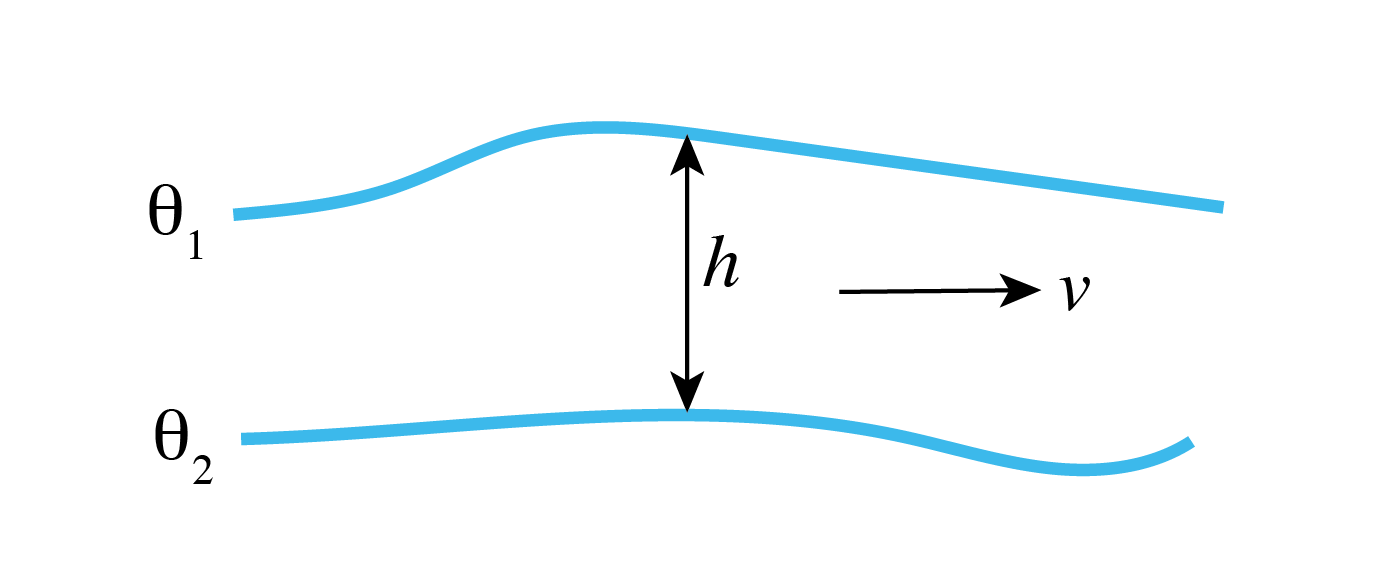Figure 4.12 Schematic of pkg/layers diagnostics.¶
43 fields(1:3,4) = 'LaVH1TH ', 'LaHs1TH ', 'LaVa1TH '
44 frequency(4) = 31104000.,
45 fileName(4) = 'layDiag',


Diagnostic LaVH1TH is the integrated meridional mass transport in the layer; here we request an annual mean time average (via the frequency parameter setting), which will effectively output the quantity $$\overline{vh}$$ (m2 s-1). LaHs1TH is the layer thickness $$h$$ (m) calculated at “v” points (see Section 2.11.4). LaVa1TH is the layer average meridional velocity $$v$$ (m/s). These diagnostics are all 3-D fields, albeit the vertical dimension here is the layer discretization in temperature space, which was defined in data.layers. See Section 4.3.5.1 for examples using these diagnostics to calculate the residual circulation and the meridional overturning circulation in density coordinates.

##### DIAG_STATIS_PARMS - Diagnostic Per Level Statistics¶

Here we specify statistical diagnostics of potential temperature and surface relaxation heat flux, output every ten days, to assess how well the model has equilibrated. See tutorial Baroclinic Ocean Gyre for a more complete description of syntax and output produced by these diagnostics.

#### 4.3.3.2.7. File input/eedata¶

This file uses standard default values (single-threaded) and does not contain customizations for this experiment.

#### 4.3.3.2.8. File input/bathy.50km.bin¶

This is a 2-D($$x,y$$) map of bottom bathymetry, as generated by the MATLAB program verification/tutorial_reentrant_channel/input/gendata.50km.m or the Python script verification/tutorial_reentrant_channel/input/gendata.50km.py. Input files are 32-bit single precision, by default. Our bathymetry file has active ocean grid cells along both the eastern and western boundaries (i.e., no land points or walls are present along either boundary), and thus our model will be fully zonally reentrant. While our northern boundary also consists entirely of active ocean points, we prescribe a wall along the southern end of our model domain, therefore the model is NOT meridionally reentrant.

Unlike in previous examples, where the bathymetry was discretized to match depths of defined vertical grid faces (rF, see Figure 2.9), we have a more complicated bottom bathymetry as defined using a sine function for our bottom ridge. The model default in such case is to round the bathymetry up or down to the nearest allowed vertical cell face level. However, the model permits the use of “partial cells” (sometimes also referred to as “shaved” or “lopped” cells), which can provide dramatic improvements in model solution (see Adcroft et al. 1997 [AHM97]). Here, we activate partial cells though parameter choices hFacMin and hFacMinDr in input/data, as discussed above. The fraction of a vertical cell that contains fluid is represented in the 3-D output variable hFacC, which will have a value of 0.0 beneath the ocean floor (and at land points), 1.0 at an active full-depth ocean cell, and a number between hFacMin and 1.0 for a partial ocean cell. As such, hFacC is often quite useful as a “mask” when computing diagnostics using model output.

As an example, consider horizontal location (10,15) in out setup here, located in our bottom ridge along the sloping notch. In our bathymetry file, the vertical level is specified as -2382.3 m. This falls between vertical faces located at -2360.1 and -2504.0 [these are grid variable rF(39:40)]. Thus, this grid cell will be included in the active ocean domain as a thin, yet legal, partial cell: hFacC(10,15,39)=0.154.

#### 4.3.3.2.9. File input/zonal_wind.50km.bin, input/SST_relax.50km.bin¶

These files are 2-D($$x,y$$) maps of zonal wind stress $$\tau_{x}$$ (Nm–2) and surface relaxation temperature (oC), as generated by program verification/tutorial_reentrant_channel/input/gendata.50km.m or verification/tutorial_reentrant_channel/input/gendata.50km.py. Note that a 2-D($$x,y$$) file is expected even though as specified, both $$\tau_{x}$$ and SST field are only $$f(y)$$.

#### 4.3.3.2.10. File input/temperature.50km.bin¶

This file specifies a 3-D($$x,y,z$$) map of temperature (oC), as generated by verification/tutorial_reentrant_channel/input/gendata.50km.m or verification/tutorial_reentrant_channel/input/gendata.50km.py (see Figure 4.11). Note again a 3-D($$x,y,z$$) file is expected despite temperature begin only $$f(y,z)$$. This file is used here for two purposes: first, as specified in input/data, these values are used for temperature initial conditions; secondly, this file was also specified in input/data.rbcs as a 3-D field used for temperature relaxation purposes.

#### 4.3.3.2.11. File input/T_relax_mask.50km.bin¶

This file specifies a 3-D($$x,y,z$$) mask, as required by /pkg/rbcs to inform the model which gridpoints to relax. These values should be between 0.0 and 1.0, with 0.0 for no restoring, 1.0 for full restoring, with fractional values as a multiplicative factor to effectively weaken restoring at that location (see Section 8.3.2). Here, we select a value of 1.0 along the model northern wall for all sub-surface depths (relaxation at the surface is specified using input/SST_relax.50km.bin, otherwise you would be restoring the surface layer twice), and use a fractional value for the $$xz$$ plane of grid cells just south of the northern border (see verification/tutorial_reentrant_channel/input/gendata.50km.m or verification/tutorial_reentrant_channel/input/gendata.50km.py).

## 4.3.4. Building and running the model¶

This model can be built and run using the standard procedure described in Section 3.5 and Section 3.6. (see also README).

For testing purposes the model is set to run 10 time steps. For a reasonable solution, we suggest running for 30 years, which requires changing nTimeSteps to 933120. When making this edit, also change monitorFreq to something more reasonable, say 10 days (=864000.). Using a single processor core, it should take 12 hours or so to run 30 years; to speed this up using MPI, re-compile using nPy=4, and nSy=1, in SIZE.h and recompile with the -mpi flag (see Section 3.6.1 for instructions how to run using MPI, here you will be using 4 cores). As an exercise, see if you can speed it up further using additional processor cores, e.g., by decreasing the tile size in $$x$$ and increasing nPx.

As configured, the model runs with pkg/gmredi activated, i.e., useGMRedi=.TRUE. in data.pkg. In Section 4.3.5.1 we will also examine a model solution using old-fashioned large horizontal diffusion with pkg/gmredi deactivated. The same executable can be used for the non-GM run. Set useGMRedi=.FALSE. in data.pkg, and also set diffKhT=1000. in data namelist PARM01. Also, comment out the lines for diagnostics list 5 in data.diagnostics or you will get (non-fatal) warning messages in STDERR.

In Section 4.3.5.2 we will present results with the resolution increased by an order of magnitude, eddy-permitting. Additional required changes to the code and parameters are discussed.

## 4.3.5. Model Solution¶

Our primary focus in this section is physical interpretation of the model solution, not how to generate plots from MITgcm output, and thus in parallel we strongly recommend carefully going through our Python analysis code, documented in Jupyter Notebook format, see verification/tutorial_reentrant_channel/analysis/py_notebook.ipynb. This notebook reads in grid data, discusses (and plots) the setup and forcing data files in additional detail, and generates figures shown in the tutorial. MATLAB analysis code to generate tutorial output figures is available at verification/tutorial_reentrant_channel/analysis/matlab_plots.m.

### 4.3.5.1. Coarse Resolution Solution¶

Before examining the circulation and temperature structure of the solution, let’s first assess whether the solution is approaching a quasi-equilibrium state after 30 years of integration. Typically, one might expect a solution given this setup to equilibrate over a timescale of a hundred years or more, given the depth of the domain and the prescribed weak vertical diffusivity. As in tutorial Baroclinic Ocean Gyre, we will make use of the ‘Diagnostic Per Level Statistics’ to assess equilibrium; specifically, we will look at the change in surface (restoring) heat flux over time, as well as the potential temperature field. In this tutorial we use standard native Fortan (binary) output files (using pkg/mdsio) rather than netCDF output (as done in tutorial Baroclinic Ocean Gyre). Important note: when using pkg/mdsio, the statistical diagnostics output is written in plain text, NOT binary format. An advantage is that this permits a simple unix cat or more command to display the file to the terminal window as integration proceeds, i.e., for a quick check that results look reasonable. The disadvantage however is that some additional parsing is required (when using MATLAB) to generate some plots using these data. Making use of MITgcm shell script utils/scripts/extract_StD, in a terminal window (in the run directory) type

% ../../../utils/scripts/extract_StD dynStDiag.0000000000.txt STATDIAGS  dat


where dynStDiag.0000000000.txt is the name of our statistical diagnostics output file, STATDIAGS is a name we chose for files generated by running the script, with extension dat. This shell script extracts data into the following (plain text) files:

• STATDIAGS_Iter.dat - list of iteration numbers for which statdiags dumped

• STATDIAGS_THETA.dat - statdiags for field THETA (diagnostic field specified in input/data.diagnostics)

• STATDIAGS_TRELAX.dat - statdiags for field TRELAX (diagnostic field specified in input/data.diagnostics)

The files STATDIAGS_Iter.dat and STATDIAGS_«DIAGNAME».dat are simple column(s) of data that can be loaded or read in as an array of numbers using any basic analysis tool. Here we will make use of another MITgcm utility, utils/matlab/Read_StD.m, which uses MATLAB to make life a bit more simple for reading in all statistical diagnostic data. In a MATLAB session type

>> [nIter,regList,time,stdiagout,listFlds,listK]=read_StD('STATDIAGS','dat','all_flds');


where

• nIter = number of iterations (i.e., time records) dumped

• regList = list of region numbers (=0 here, as we did not define any regions, by default global output only)

• time(:,1) = iteration numbers ; time(:,2) = time in simulation (seconds)

• listFlds = list of fields dumped

• listK = for each field, lists number of k levels dumped

• stdiagout = 5 dimensional output array ( kLev, time_rec, region_rec, [ave,std,min,max,vol], fld_rec ) where kLev=1 is depth-average, kLev=2:50 is for depths rC(1:49)

A function to parse statistical diagnostics MITgcm output is also available in the python package MITgcmutils. Executing the python command

stdiags_bylev,stdiags_2D,iters = readstats('dynStDiag.0000000000.txt')


will load up the level-by-level statistical diagnostics into stdiags_bylev (e.g., stdiags_bylev['THETA'][:,0,0] is the time series for top level average temperature), stdiags_2D given column-integrated or 2-D fields (e.g., stdiags_2D['TRELAX'][:,0] is the time series for surface heat flux), and iters is iteration number for the time series (e.g. iters['TRELAX'] is a series of iteration numbers for the THETA diagnostic, the user is left to convert into time units). See the MITgcmutils documention for more information.

On the left side of Figure 4.13 we show time series of global surface heat flux. In the first decade there is rapid adjustment, with a much slower trend in both mean and standard deviation in years 10-30. In the mean there remains a significant heat flux into the ocean in the run without GM (solid), whereas with GM (dashed) the net heat uptake is also positive, but smaller. The panels on the right show potential temperature at the surface, mid-level (270 m) and at depth. Note in particular the warming trend at depth in the run without GM. The SST series display a much less obvious trend (as might be expected given rapid restoring of SST). Examining these results, we see that after 30 years our run is not at full equilibrium, presumably due to the long timescale for vertical diffusion. And, we infer that less surface heating is penetrating to depth in the GM solution. This difference is also obvious in Figure 4.14 where we plot zonal mean temperature: note the deeper thermocline in the left panel (without GM), in addition to the deeper mixed layer (and warmer surface) in the southern half of the model domain. The differences in convective adjustment are remarkable, as shown in Figure 4.15; here we plot a plan view of diagnostic CONVADJ, which is the fraction of the time steps a grid cell is convectively unstable, at 92 m depth. Note that at this depth, convection is limited to grid cells near the southern boundary in the GM run, whereas a significant portion of the domain is convecting in the non-GM run: as discussed in Gent (2011) [Gen11], the Deacon cell advects cold water northward at the surface, resulting in unstable water columns and excessively deep mixed layers. Clearly, the temperature structure of the model solution is sensitive to our mesoscale eddy parameterization (we will explore this further).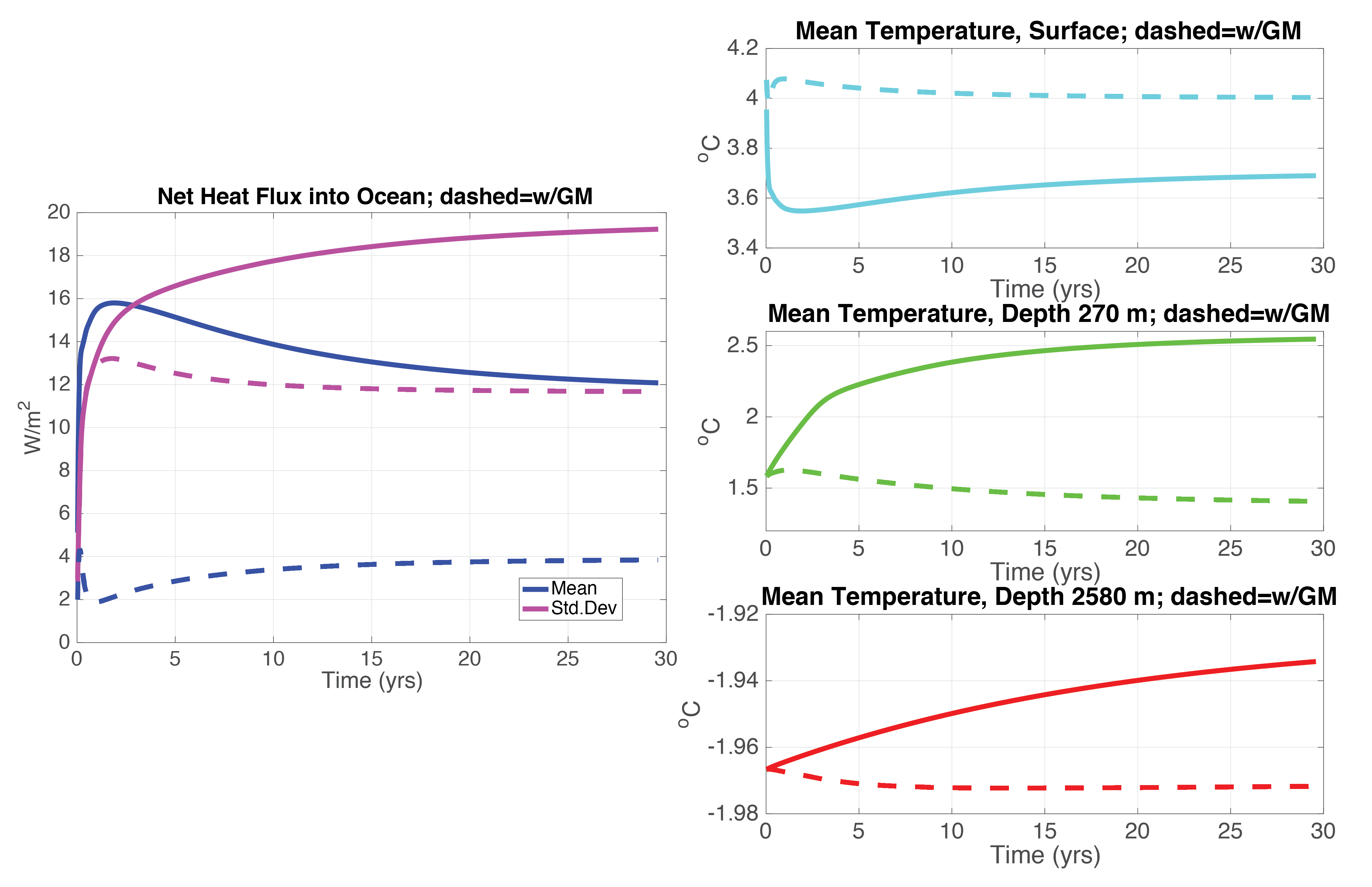Figure 4.13 Left: time series of area-integrated heat flux into the surface ocean (blue) and its standard deviation (magenta). Right: area-mean temperature at the surface (top, cyan), in the thermocline (middle, green), and at depth (bottom, red). In all panels, solid curves show non-GM run, dashed curves include GM.¶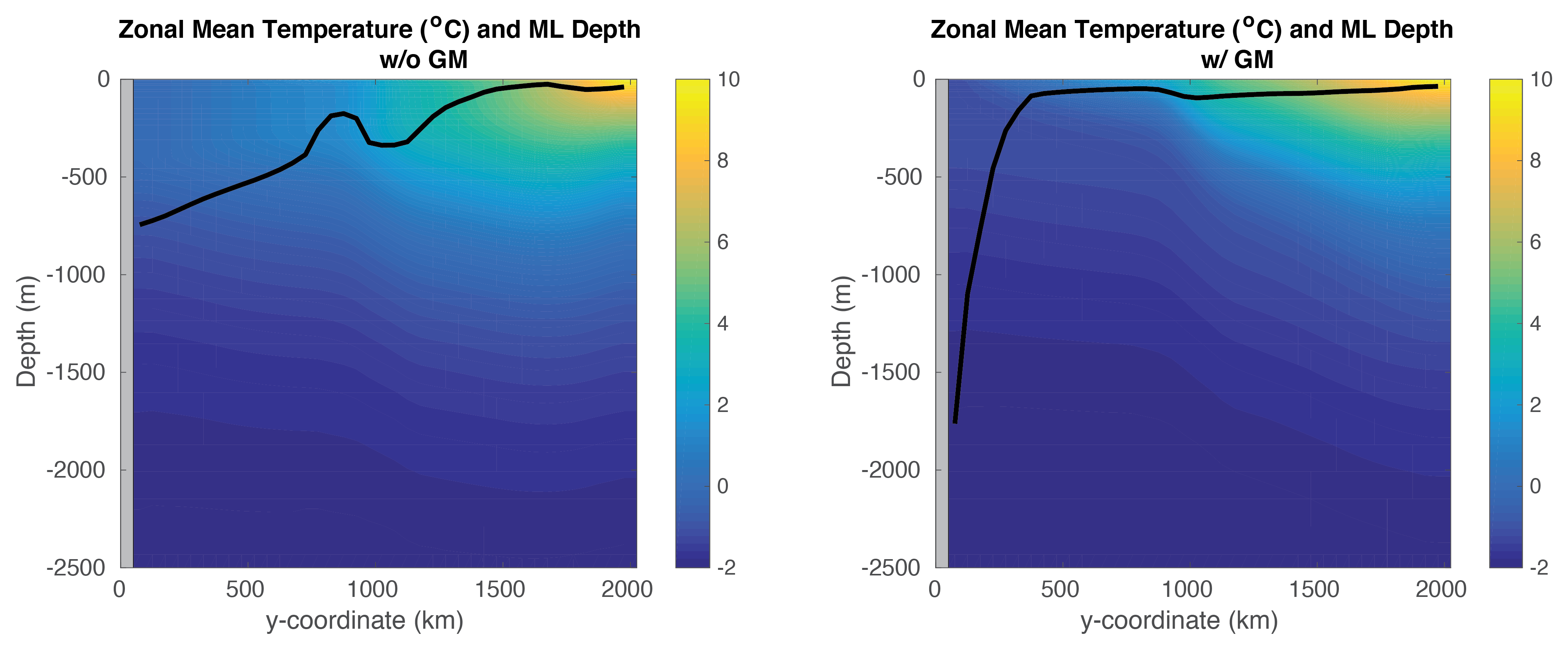Figure 4.14 Zonal-mean temperature (shaded) and zonal-mean mixed layer depth (black line) averaged over simulation year 30. Left plot is from non-GM run, right using GM.¶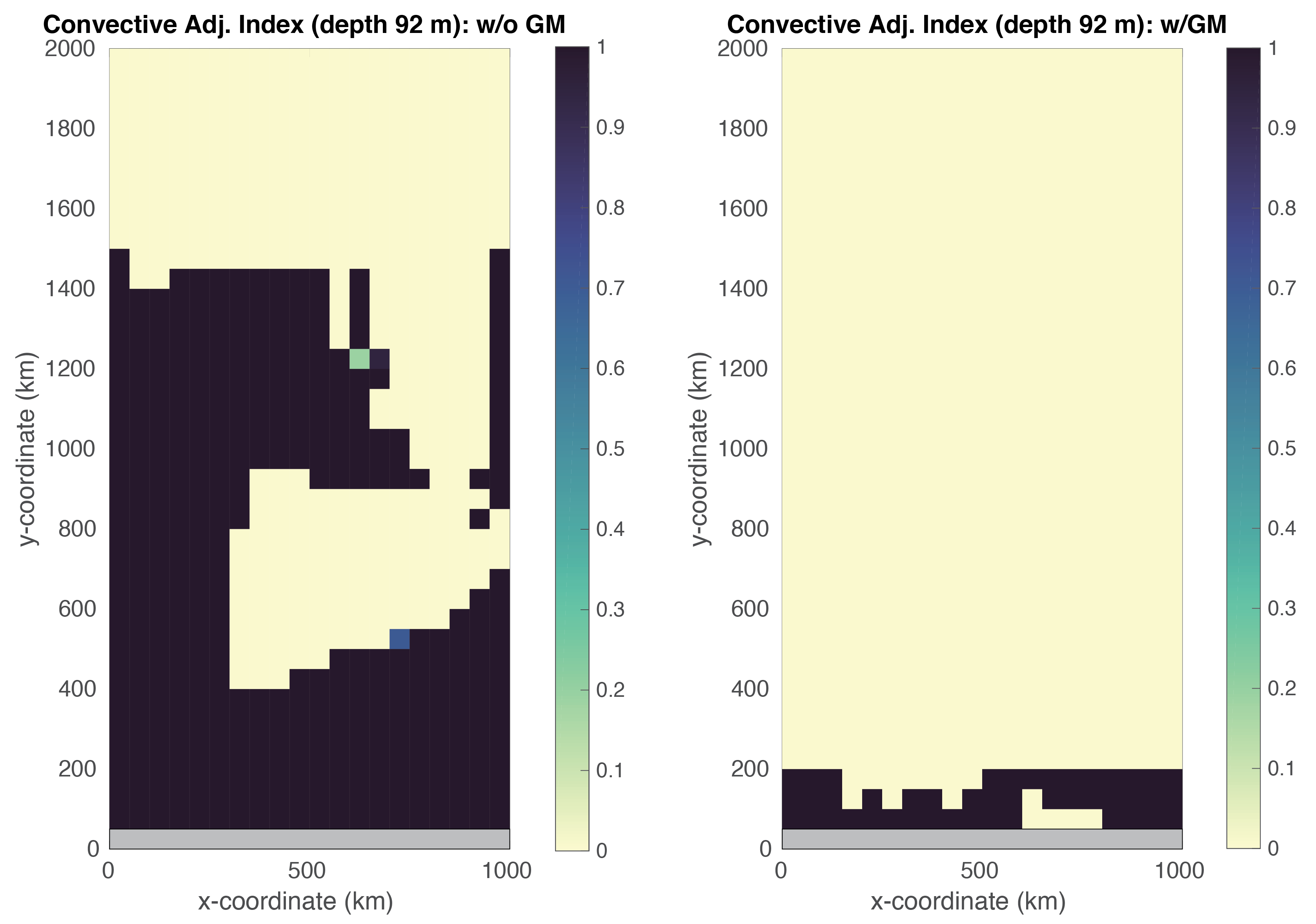Figure 4.15 Convective adjustment index: 0= never convectively unstable during year 30, 1= always convectively unstable. Left plot is from non-GM run, right using GM.¶

Figure 4.16 shows the barotropic streamfunction without GM (left) and with GM (right). The pattern is quite similar in both simulations, characterized by a jet centered in the latitude bands with the deep notch, with some deflection to the south after the jet squeezes through the notch. There is a balance between negative relative vorticity, as the jet curves northward through the notch and then southward again, and increasing $$f$$ to the north (from the beta-plane) such that barotropic potential vorticity is conserved. North of the notch, we see in Figure 4.14 the ocean is much more stratified, with dynamics presumably more baroclinic.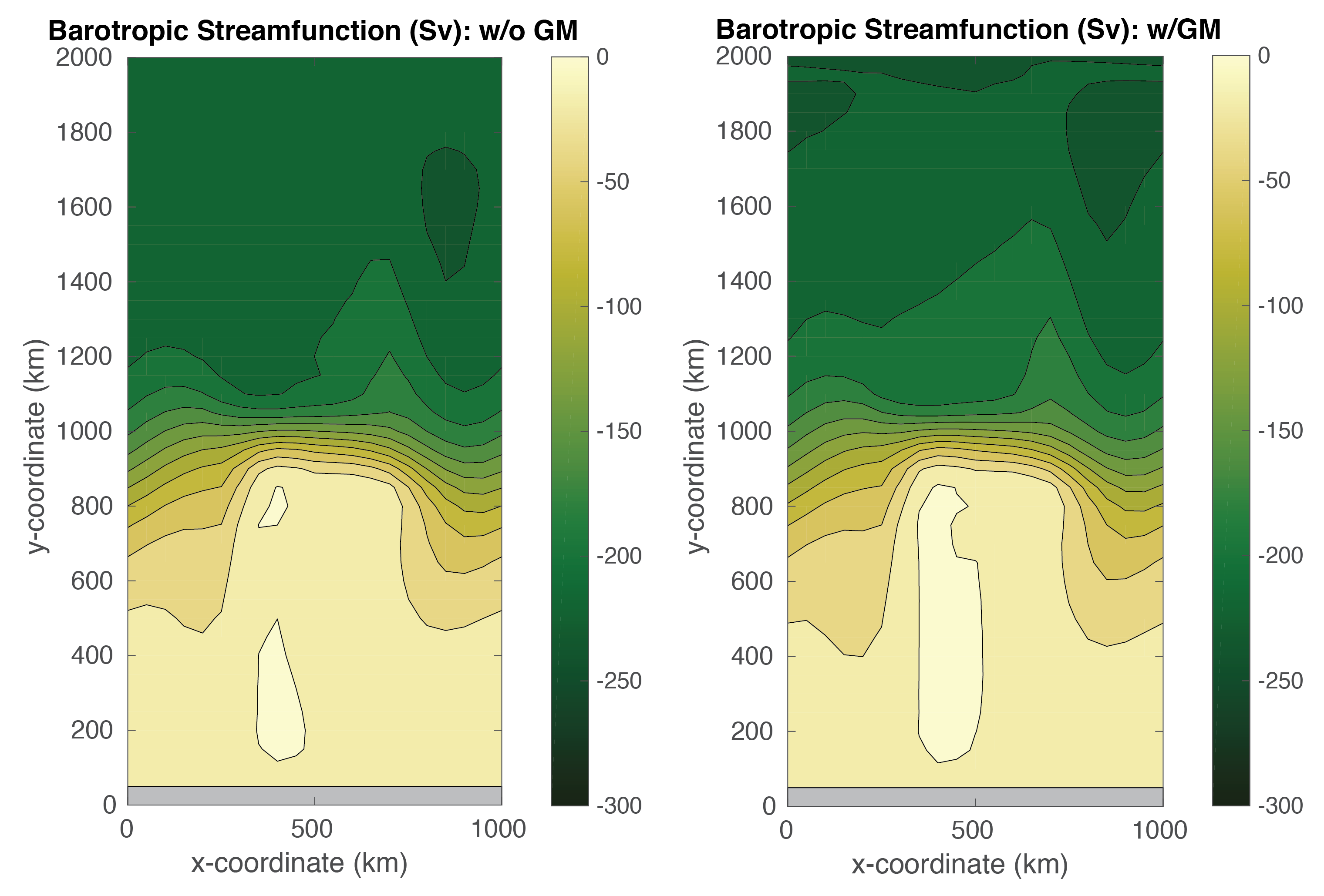Figure 4.16 Barotropic streamfunction averaged over over simulation year 30. Left plot is from non-GM run, right using GM. Contour interval is 20 Sv.¶

Figure 4.17 shows the Eulerian meridional overturning circulation for the non-GM run (left) and GM run (right). Again, they appear quite similar; what we are observing here is known as a “Deacon Cell” (Deacon 1937 [Dea37]; Bryan 1991 [Bry91]) forced by surface Ekman transport to the north (see also Döös and Webb 1994 [DW94], Speer et al. 2000 [SS00]), with downwelling in the northern half of the basin and upwelling in the south. The magnitude of this cell, on the order of 1-2 Sverdrups, may not seem very impressive, but it is important to consider our zonal domain spans only about 1/20th of the 60th parallel south; scaled up, the magnitude of this cell is quite large. Some local recirculation occurs in the latitude bands where the ridge slopes down to the center of the deep notch. The centers of these recirculations occur in the bottom 2000 m, where stratification is quite weak, so much of water recirculated here falls within a very narrow density class. The deep ridge effectively creates east-west sidewalls at depth, thus able to support an overturning in thermal wind balance, whereas no sidewalls exist in the upper portion of the water column. There is little overturning associated with the deep jet flowing through the flat bottom of the notch.

Also worth noting is that we see some evidence of noise (jaggedy contours) in Figure 4.17, despite our rather large choice of $$A_{h}$$=2000 m2 s–1 for (uniform) horizontal viscosity and our higher-order advective scheme. These noise artifacts increase fairly dramatically for smaller choices of $$A_{h}$$, although we tested the solution remains stable for $$A_{h}$$ decreased by an order of magnitude.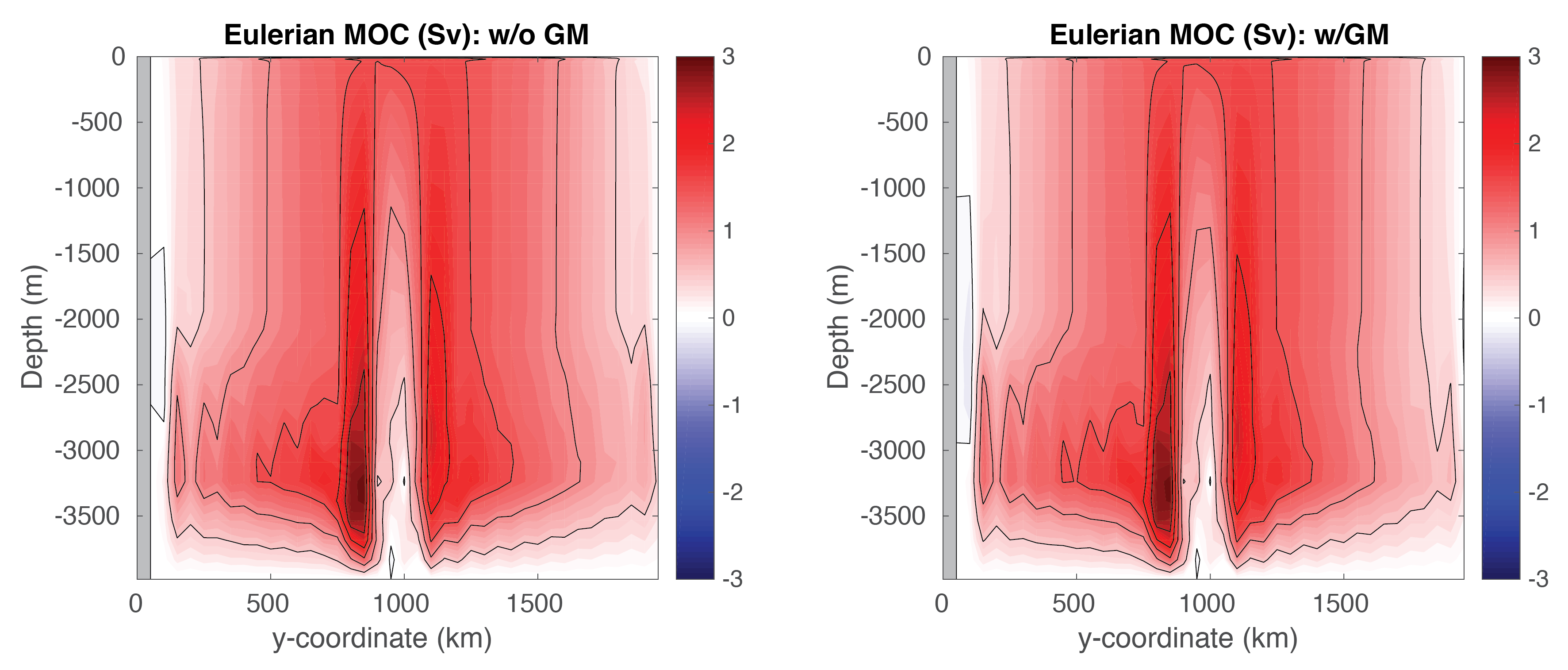Figure 4.17 Eulerian meridional overturning circulation (shaded) averaged over simulation year 30. Left plot is from non-GM run, right using GM. Contour interval is 0.5 Sv.¶

When using pkg/gmredi, it is often desirable to diagnose an eddy bolus velocity, or a bolus transport, in order to compute the residual circulation (Ferrari 2003 [FP03]), the Lagrangian transport in the ocean (i.e., which effects tracer transport; see, for example, Wolfe 2014 [Wol14]). Unfortunately the bolus velocity is not directly available from MITgcm, but must be computed from other GM diagnostics, which differ if the skew flux or bolus/advective form of GM is selected. Here we choose the later form in data.gmredi (GM_AdvForm =.TRUE.), for which a bolus streamfunction diagnostic is available, thus the bolus velocity can be readily computed (see matlab_plots.m; obtaining the bolus velocity, for reasons of gridding, is a bit more straightforward using the advective form). In Figure 4.18 we’ve computed and added the bolus velocity to the Eulerian velocity. We see that the upper meridional overturning cell has weakened in magnitude, particularly in the northern half of the domain. The eddy parameterization will attempt to flatten sloping isopycnals seen in Figure 4.14, creating a bolus overturning circulation in the opposite sense to the Deacon Cell. The magnitude of the GM thickness diffusion effectively controls the strength of the eddy transport; here we observed only partial cancellation of the Deacon Cell shown in Figure 4.17. In global ocean general circulation models, an observation of near-cancellation in the Southern Ocean Deacon Cell when the GM parameterization was used was first reported in Danabasoglu et al. (1994) [DMG94].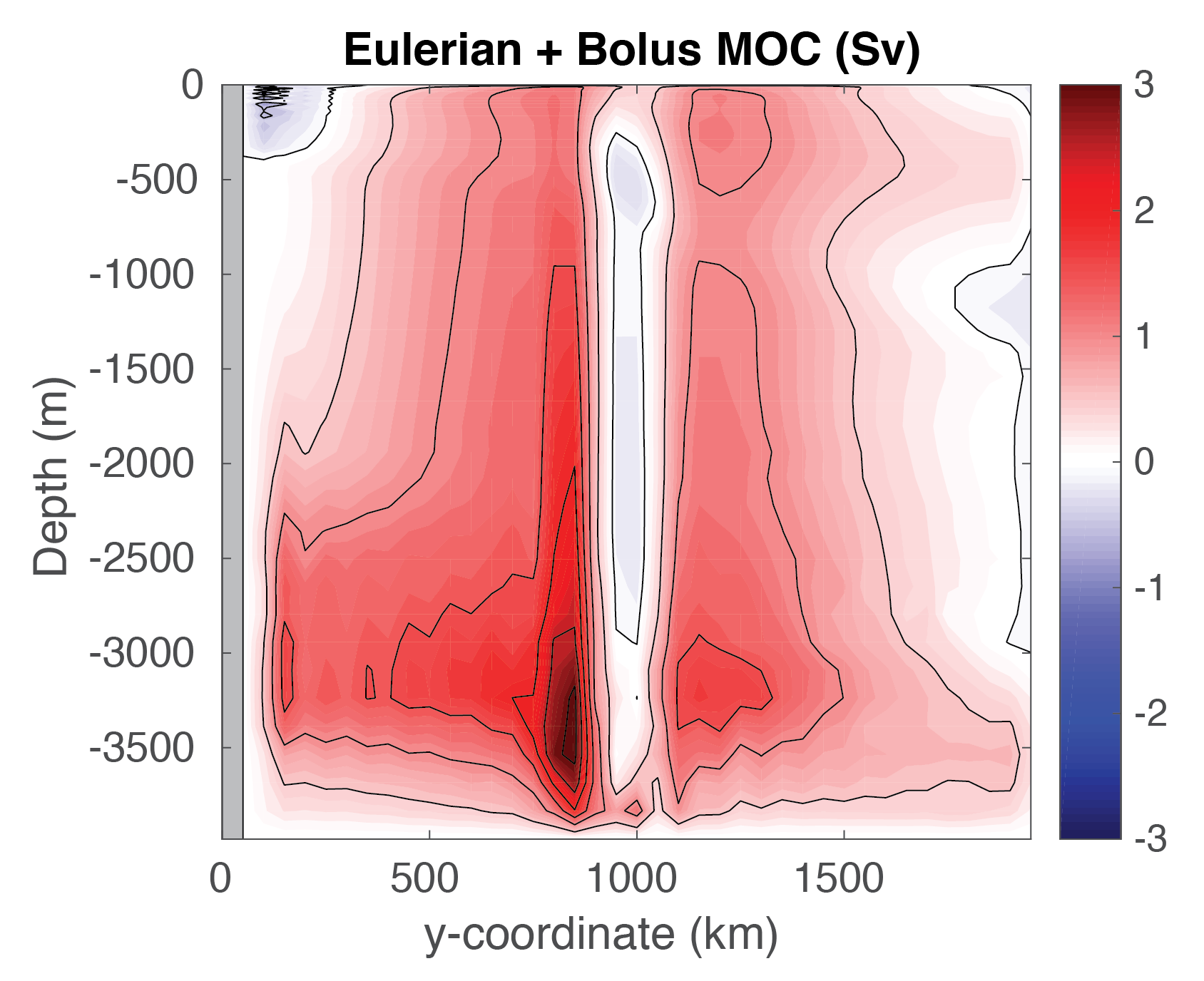Figure 4.18 Meridional overturning circulation (shaded) from GM simulation including bolus advective transport, averaged over simulation year 30. Contour interval is 0.5 Sv.¶

Now let’s use pkg/layers output to examine the residual meridional overturning circulation, shown in Figure 4.19. We integrate the time- and zonal-mean transport in isopycnal layers (see Figure 4.12) to obtain a streamfunction in density coordinates. See Abernathy et al. (2011) [AMF11] for a more detailed explanation of this calculation; this approach is the tried-and-true method to diagnose the residual circulation in an eddy-permitting regime, as required when we run this setup at higher resolution (Section 4.3.5.2). Note that pkg/layers automatically includes bolus transport from pkg/gmredi in its calculations, assuming GM is used. With temperature as the ordinate in Figure 4.19, vertical flows reflect diabatic processes. The green dashed lines represent the maximum and minimum SST for a given latitude band, thus representing upper layer circulation within this band. On the left side, without GM, we again see a robust Deacon cell, with a strong diabatic component, presumably due to horizontal diffusion occurring across sloping isopycnals (i.e. the so-called “Veronis effect”, see Veronis (1975) [Ver75] as well as other numerous papers prior to the wide-spread adoption of the GM parameterization in ocean models). [As an aside, it is for lack of a better name that we label this left plot of Figure 4.19, lacking either eddies or GM, as the residual circulation, as indeed it is identical to the Eulerian circulation in density coordinates]. On the right side, with GM, the Deacon cell is much weaker due to partial cancellation from the bolus circulation, as noted earlier, but also note that interior contours of streamfunction run roughly horizontal in the plot. We see some evidence of a deep cell in the lowest temperature classes, less obvious in the Eulerian MOC Figure 4.17. One might ask: what happened to the deep recirculating cells seen in Figure 4.18? Recall that our discretization of temperature layers is fairly crude, 0.25 K in the coldest temperatures, and presumably much of this recirculation is “lost” as recirculation within a single density class. If this deep circulation were of interest, one could simply re-run the model with finer resolution at depth (perhaps increasing the number of layers used, which requires changing LAYERS_SIZE.h and recompiling).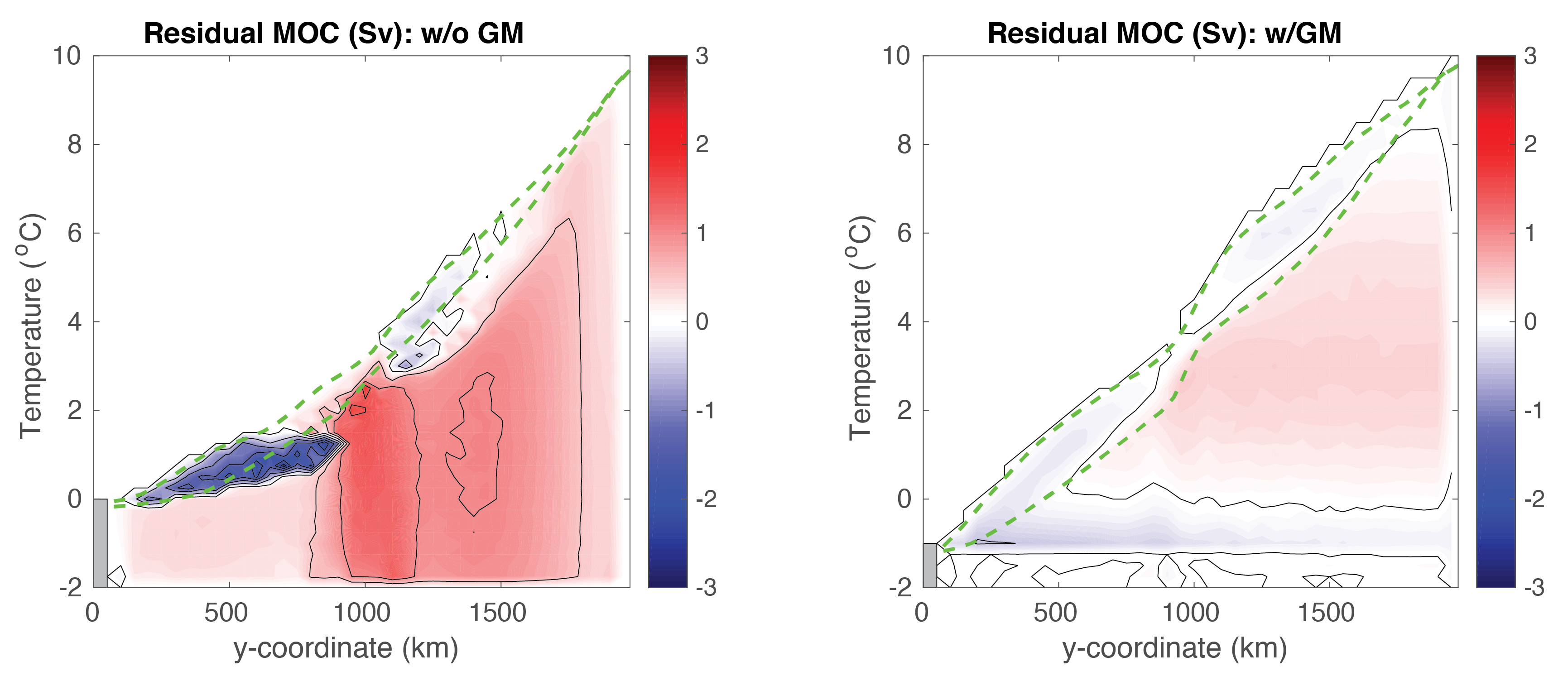Figure 4.19 Residual meridional overturning circulation (shaded) as computed in density (i.e., temperature) coordinates, averaged over simulation year 30. Contour interval is 0.5 Sv. Green dashed curves show maximum and minimum SST in each latitude band. Left plot is from non-GM run, right using GM.¶

Finally, let’s convert the residual circulatiom shown in Figure 4.19 back into depth coordinates, see Figure 4.20. Solid lines now display contours of zonal mean temperature. On the left, consistent with previous analyses, we see a small, upper ocean counter-clockwise circulation in the southern sector, where deep mixed layers occur (Figure 4.14), with the dominant feature again being the (clockwise) Deacon cell. In contrast, using GM, we see a weak residual clockwise cell aligned along temperature surfaces in the thermocline, with a weak deep counter-clockwise cell aligned with the coldest temperature contour (i.e., the deep cell seen in Figure 4.19).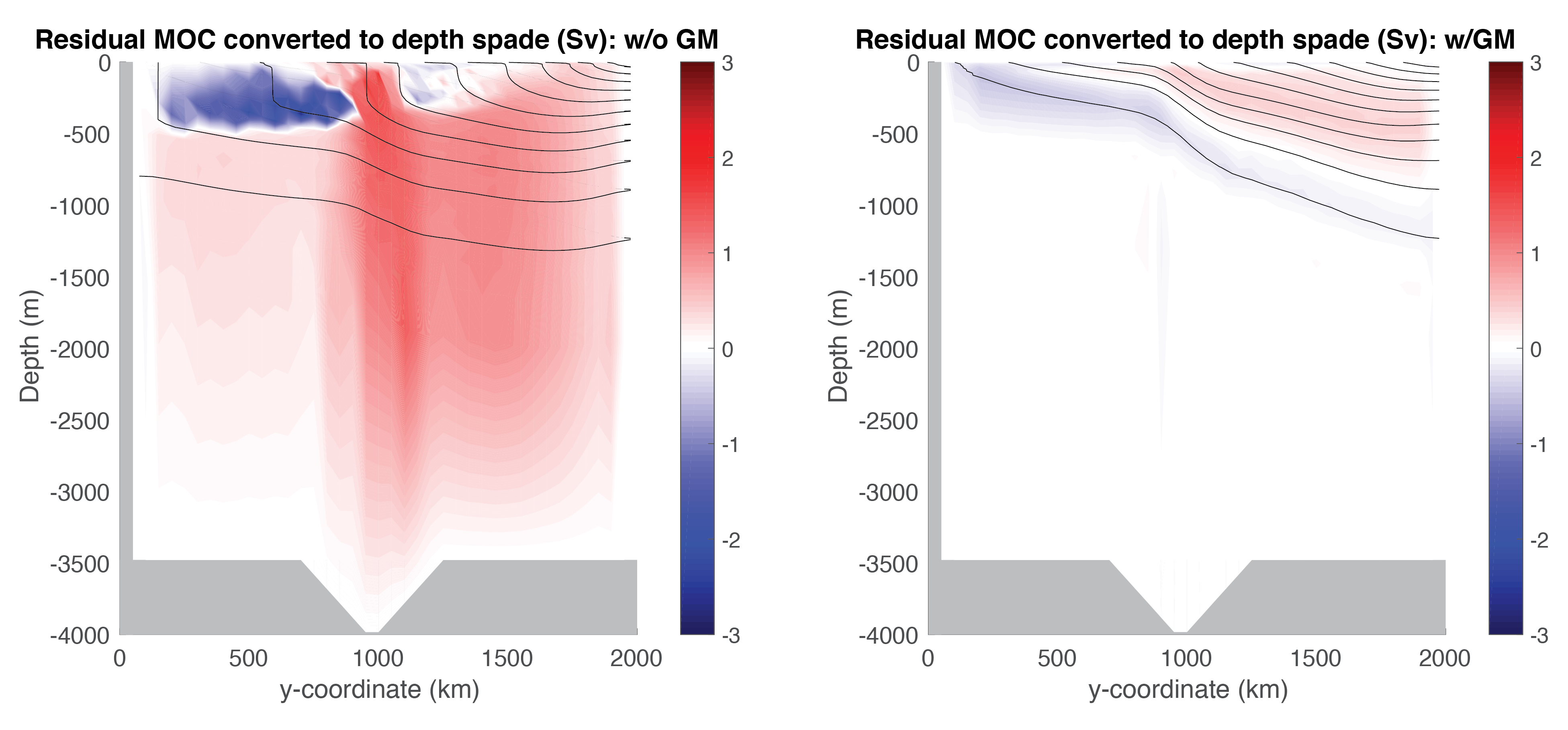Figure 4.20 Residual meridional overturning circulation (shaded) as computed in density coordinates and converted back into (zonal mean) depth coordinates, averaged over simulation year 30. Black lines show zonal mean temperature, contour interval 1 oC. Left plot is from non-GM run, right using GM.¶

### 4.3.5.2. Eddy Permitting Solution¶

In this section we discuss a model solution with the horizontal grid space reduced from 50 km to 5 km, which is sufficiently resolved to permit eddies to form (see above, which shows SST, surface relative vorticity, and surface current speed, left to right, toward the end of the 30-year simulation). Vertical resolution is unchanged. While we provide instructions on how to compile and run in this new configuration, it will require parallelizing (using MPI) on at least a hundred processor cores or else a 30-year integration will take on the order of a month or longer – in other words, this requires a large cluster or high-performance computing (HPC) facility to run efficiently.

Running with higher resolution requires re-compiling the code after changing the tile size and number of processors, see code/SIZE.h_eddy (as configured here, for 100 processors; for faster results change the tile size and use 200 or even 400 processors). Note we will NOT enable pkg/gmredi in our eddy run, so it can be eliminated from the list in packages.conf 1 (make sure to set useGMRedi=.FALSE. in data.pkg).

In conjunction with the change in code/SIZE.h_eddy, uncomment these lines in PARM04 in data:

delX=200*5.E3,
delY=400*5.E3,


to specify 5 km resolution in 200 $$\times$$ 400 grid cells in $$x$$ and $$y$$. New files for bathymetry, forcing fields, and initial temperature can be generated using the MATLAB program verification/tutorial_reentrant_channel/input/gendata_5km.m or Python script verification/tutorial_reentrant_channel/input/gendata.5km.py (don’t forget to change the filenames in data.rbcs and PARM05 in data).

Running at higher resolution requires a smaller time step for stability. Revisiting Section 4.3.2.1, to maintain advective stability (CFL condition, (4.24)) one could simply decrease the time step by the same factor of 10 decrease as $$\Delta x$$ – stability of inertial oscillations is no longer a limiting factor, given a smaller $$\Delta t$$ in (4.25) – but to speed things up we’d like to keep $$\Delta t$$ as large as possible. With a rich eddying solution, however, is it clear that horizontal velocity will remain order ~1 ms–1? As a compromise, we suggest setting parameter DeltaT=250. (seconds) in data, which we found to be stable. For this choice, a 30-year integration requires setting nTimeSteps=3732480.

While it would be possible to decrease (spatially uniform) harmonic viscosity to a more appropriate value for this resolution, or perhaps use bi-harmonic viscosity (see Section 2.14.5), we will make use of one of the nonlinear viscosity schemes described in Section 2.19, geared toward large eddy simulations, where viscosity is a function of the resolved motion. Here, we employ the Leith viscosity (Leith 1968, Leith 1996 [Lei68] [Lei96]). Set the following parameters in PARM01 of data:

viscC2Leith = 1.,
useFullLeith=.TRUE.,
viscAhGridMax = 0.5,


(and comment out the line viscAh =2000. ). viscC2Leith is a scaling coefficient which we set to 1.0, useFullLeith =.TRUE. uses unapproximated gradients in the Leith formulation (see Section 2.19.1.4). Parameter viscAhGridMax places a maximum limit on the Leith viscosity so that the CFL condition is obeyed (see Section 2.19.1.7 and (4.26) in discussion of Numerical Stability Criteria). The values of viscAh that the Leith scheme generates in this solution generally range from order 1 m2 s–1 in regions of weak flow to over 100 m2 s–1 in jets. Note that while it would have been possible to use the Leith scheme in the 50 km resolution setup, the scheme was not really designed to be used at such a large $$\Delta x$$, and the $$A_{h}$$ it generates about an order of magnitude below the constant $$A_{h} = 2000$$ m2 s–1 employed in the coarse model runs, resulting in a very noisy solution.

Finally, we suggest adding the parameter useSingleCpuIO =.TRUE. in PARM01 of data. This will produce global output files generated by the master MPI processor, rather than a copious amount of single-tile files (each processor dumping output for its specific sub-domain).

To compare the eddying solution with the coarse-resolution simulations, we need to take a fairly long time average; even in annual means there is noticeably variability in the solution. Figure 4.21 through Figure 4.23 plot similar figures as Figure 4.14-Figure 4.20, showing a time mean over the last five years of the simulation.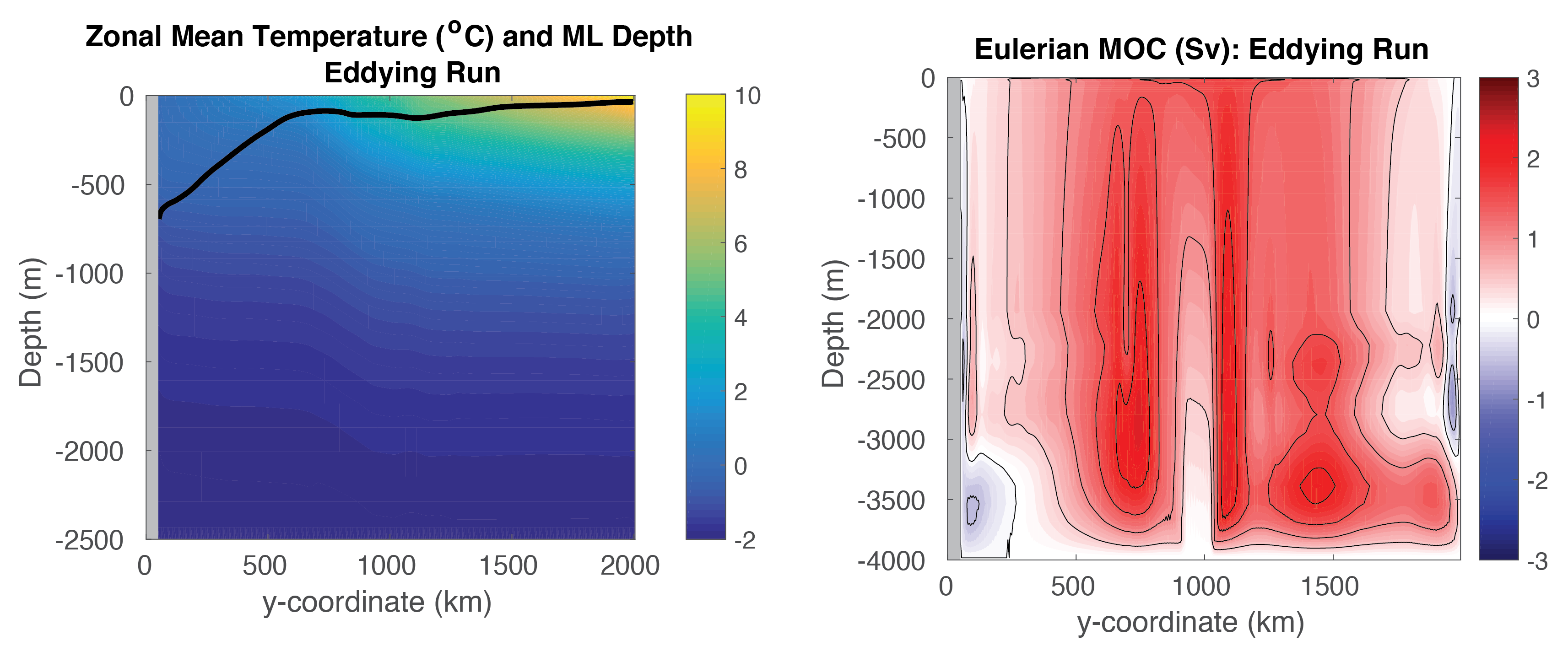Figure 4.21 Left: Zonal-mean temperature (shaded) and zonal-mean mixed layer depth (black line) from eddying simulation averaged over years 26-30. Right: Eulerian meridional overturning circulation (shaded) from eddying simulation averaged over years 26-30. Contour interval is 0.5 Sv.¶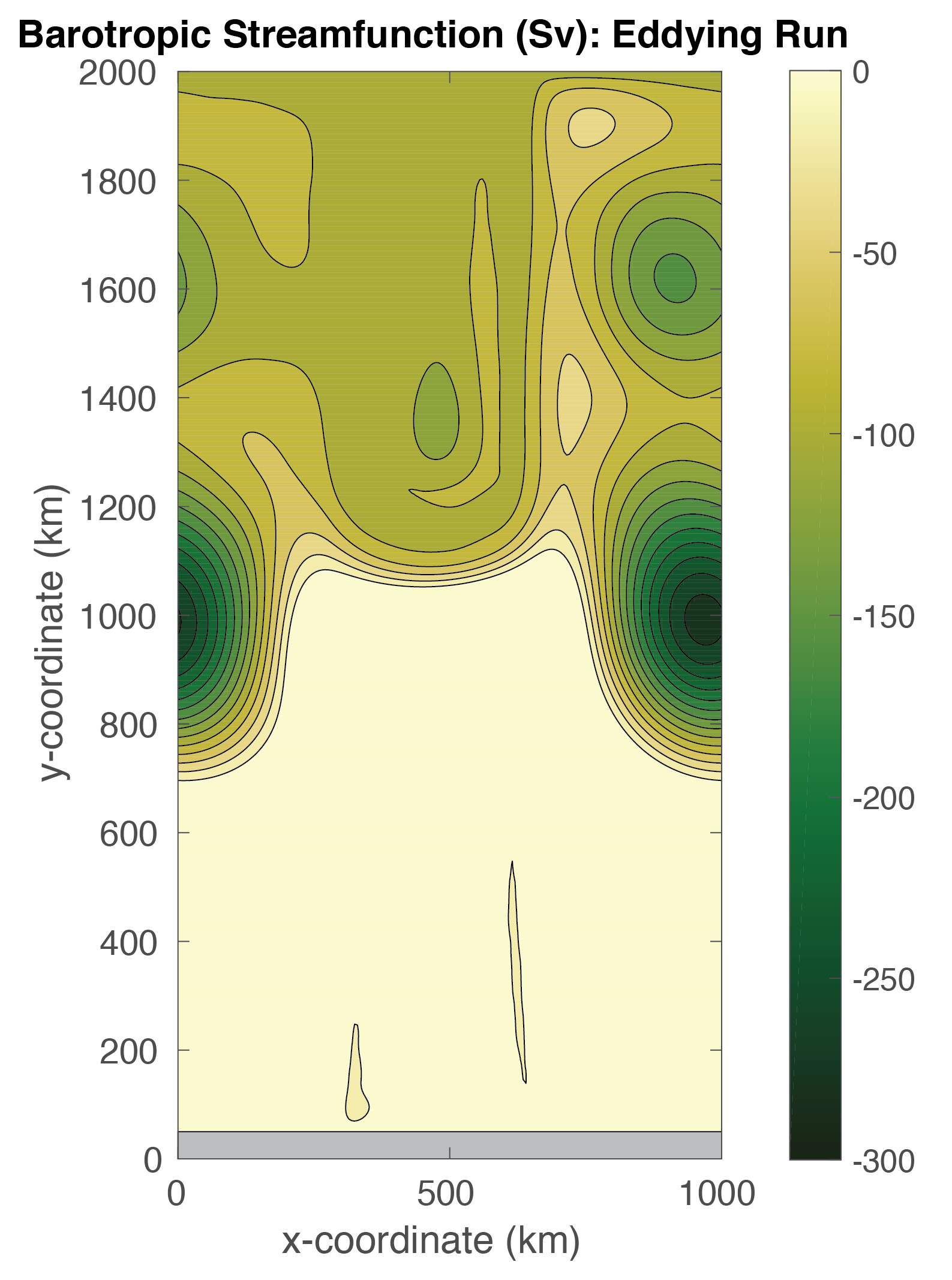Figure 4.22 Barotropic streamfunction from eddying simulation averaged over years 26-30. Contour interval is 20 Sv.¶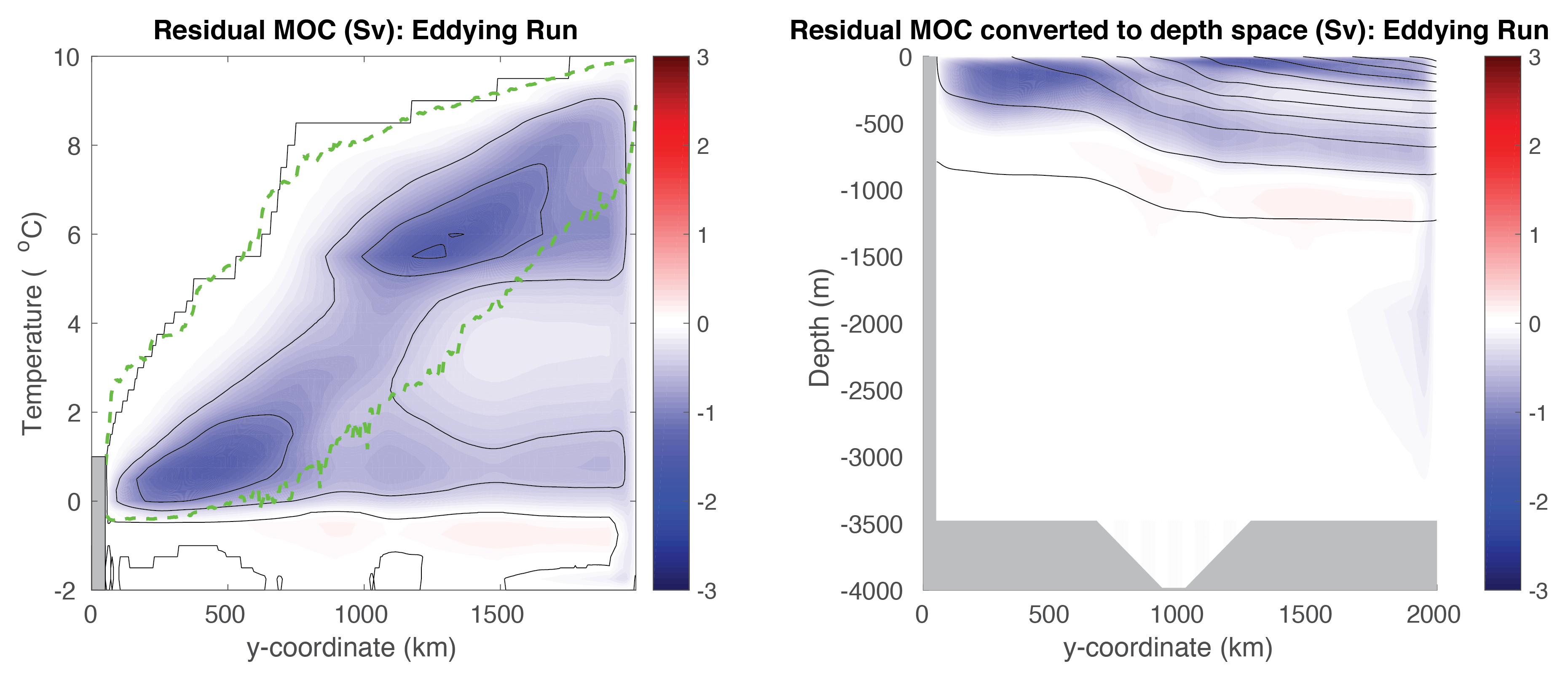Figure 4.23 Left: Residual meridional overturning circulation (shaded) as computed in density (i.e., temperature) coordinates, from eddying simulation averaged over years 26-30. Contour interval is 0.5 Sv. Green dashed curve shows maximum and minimum (instantaneous) SST in each latitude band. Right: Residual meridional overturning circulation (shaded) as computed in density coordinates and converted back into depth coordinates, from eddying simulation averaged over years 26-30. Black lines show zonal mean temperature, contour interval 1 oC.¶

In general, our coarse resolution solutions are not a bad likeness of the (time mean) eddying solution, particularly when we use pkg/gmredi to parameterize mesoscale eddies. More detailed comments comparing these solutions are as follows:

• The superiority of the GM solution is clear in the plot of zonal mean temperature (Figure 4.21 left panel vs. Figure 4.14) and the residual overturning circulation (Figure 4.23 vs. Figure 4.19 and Figure 4.20). Differences among the Eulerian MOC plots (Figure 4.21 right panel vs. Figure 4.17) are less obvious, but note that in the more stratified northern section of the domain, the eddying MOC looks more like the coarse “Eulerian + Bolus” GM solution (Figure 4.18). However, these two fields are not expected to be equal, since the eddying MOC calculated by layers also includes a stationary eddy component (Viebahn and Eden 2012 [VE12]; Dufour et al. 2012 [DSZ+12]).

• A large anticyclonic barotropic vortex is present away from the topographic ridge as shown in a plot of the barotropic streamfunction (Figure 4.22; recall, our domain is located in the Southern Hemisphere, so anticyclonic is counter-clockwise). As such, the flow passing through the deep notch is somewhat less than obtained in the coarse solution (Figure 4.16). Yet, similar constraints on barotropic potential vorticity conservation lead to a similar overall pattern.

• Examining the residual circulation generated from pkg/layers diagnostics (see Figure 4.23 vs. Figure 4.19, Figure 4.20), the non-GM solution seems quite poor, which would certainly have implications on tracer transport had any additional tracers been included in the simulation. In the GM solution, eddies seem to only partially cancel the cell forced by northward Ekman transport (Deacon Cell). In the eddying solution, the residual circulation is oriented in the opposite sense: eddy fluxes resulting from baroclinic instability due to the northern sponge layer (stratification) overwhelms the Deacon Cell. This would seem to suggest than our parameterization of eddies by GM, or more specifically, our choice for parameter GM_background_K of 1000 m2 s–1, may be too low, at least for this idealized setup! Parameterizing eddies in the Southern Ocean is a topical research question, but some studies suggest this value of GM thickness diffusivity may indeed be low for values in the Southern Ocean (e.g., Ferriera et al. 2005 [FMH05]). A weak residual deep cell, oriented with rising flow along the sponge layer, is also present. Note that the area enclosed by the dashed green lines in Figure 4.23 is quite large, due to episodic large deviations in SST associated with eddies.

• As might be suggested by the orientation of the residual MOC, in the eddying solution temperature relaxation in the sponge layer is associated with heat gain in the thermocline. In the coarse runs, however, the sponge layer is effectively cooling, particularly in the non-GM run. Although at present there is no diagnostic available in pkg/rbcs which directly tabulates these fluxes, computing them is quite simple: the heat flux (in watts) into a grid cell in the sponge layer is computed as $$\rho \text{C}_p {\cal V}_\theta * \frac{\theta (i,j,k) - \theta_{\rm rbc} (i,j,k)}{\tau_T} * M_{\rm rbc}$$ where $$\text{C}_p$$ is HeatCapacity_Cp (3994.0 J kg–1 K–1 by default), $${\cal V}_\theta$$ is the grid cell volume (rA(i,j) * drF(k) * hFacC(i,j,k); see Section 4.3.3.2.8 for definition of hFacC), $$\theta (i,j,k)$$ is gridpoint potential temperature (oC), $$\theta (i,j,k)_{\rm rbc}$$ is gridpoint relaxation potential temperature (oC, as prescribed in file input/temperature.5km.bin or input/temperature.50km.bin), $$\tau_T$$ is the restoring timescale tauRelaxT (as set in data.rbcs to 864,000 seconds or 10 days), and $$M_{\rm rbc}$$ is a 3-D restoring mask (values between 0.0 and 1.0 as discussed above) as specified in file T_relax_mask.5km.bin or T_relax_mask.50km.bin.

1

Note it is not stricly necessary to remove pkg/gmredi from your high-resolution build – however, if kept in the list of packages included in packages.conf, it then becomes necessary to deactivate in data.pkg for this run by setting useGMRedi=.FALSE.. If by chance you set a use«PKG» flag to .TRUE. in data.pkg but have not included the package in the build, the model will terminate with error on startup. But you can alway set a use«PKG» flag to .FALSE. whether or not the package is included in the build.